# JP4197482B2 - Base station transmission method, base station transmission apparatus, and communication terminal - Google Patents

Base station transmission method, base station transmission apparatus, and communication terminal Download PDF

## Info

Publication number
JP4197482B2
JP4197482B2 JP2003366249A JP2003366249A JP4197482B2 JP 4197482 B2 JP4197482 B2 JP 4197482B2 JP 2003366249 A JP2003366249 A JP 2003366249A JP 2003366249 A JP2003366249 A JP 2003366249A JP 4197482 B2 JP4197482 B2 JP 4197482B2
Authority
JP
Japan
Prior art keywords
signal
transmission
antenna
reception
Prior art date
Legal status (The legal status is an assumption and is not a legal conclusion. Google has not performed a legal analysis and makes no representation as to the accuracy of the status listed.)
Active
Application number
JP2003366249A
Other languages
Japanese (ja)
Other versions
JP2004254285A (en
JP2004254285A5 (en
Inventor

Original Assignee
パナソニック株式会社
Priority date (The priority date is an assumption and is not a legal conclusion. Google has not performed a legal analysis and makes no representation as to the accuracy of the date listed.)
Filing date
Publication date
Priority to JP2002329453 priority Critical
Priority to JP2002374393 priority
Priority to JP2003018761 priority
Application filed by パナソニック株式会社 filed Critical パナソニック株式会社
Priority to JP2003366249A priority patent/JP4197482B2/en
Publication of JP2004254285A publication Critical patent/JP2004254285A/en
Publication of JP2004254285A5 publication Critical patent/JP2004254285A5/ja
Application granted granted Critical
Publication of JP4197482B2 publication Critical patent/JP4197482B2/en
Active legal-status Critical Current
Anticipated expiration legal-status Critical

• 230000005540 biological transmission Effects 0.000 title claims description 364
• 238000004891 communication Methods 0.000 title claims description 58
• 230000000051 modifying Effects 0.000 claims description 212
• 239000011159 matrix materials Substances 0.000 claims description 162
• 239000000969 carriers Substances 0.000 claims description 113
• 230000001702 transmitter Effects 0.000 claims description 7
• 230000001276 controlling effects Effects 0.000 claims description 3
• 238000004364 calculation methods Methods 0.000 description 114
• 239000000203 mixtures Substances 0.000 description 100
• 230000000875 corresponding Effects 0.000 description 44
• 238000010586 diagrams Methods 0.000 description 39
• 238000000926 separation method Methods 0.000 description 34
• 238000000034 methods Methods 0.000 description 29
• 238000006243 chemical reactions Methods 0.000 description 24
• 230000002194 synthesizing Effects 0.000 description 23
• 230000015572 biosynthetic process Effects 0.000 description 19
• 238000003786 synthesis reactions Methods 0.000 description 19
• 238000007476 Maximum Likelihood Methods 0.000 description 8
• 239000011575 calcium Substances 0.000 description 7
• 239000010955 niobium Substances 0.000 description 6
• 238000005562 fading Methods 0.000 description 4
• 230000007274 generation of a signal involved in cell-cell signaling Effects 0.000 description 4
• 238000001228 spectrum Methods 0.000 description 4
• 238000001308 synthesis method Methods 0.000 description 4
• 239000000562 conjugates Substances 0.000 description 3
• 238000009792 diffusion process Methods 0.000 description 3
• 230000000694 effects Effects 0.000 description 3
• 238000010187 selection method Methods 0.000 description 3
• 238000004088 simulation Methods 0.000 description 3
• 230000003044 adaptive Effects 0.000 description 1
• 239000002131 composite materials Substances 0.000 description 1
• 238000005516 engineering processes Methods 0.000 description 1
• 230000003595 spectral Effects 0.000 description 1
• 230000001131 transforming Effects 0.000 description 1

## Images

•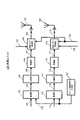•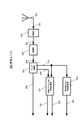•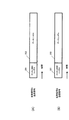•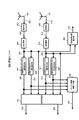•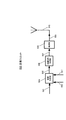•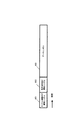•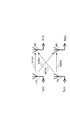•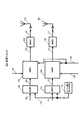•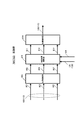•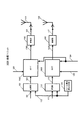•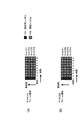••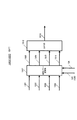•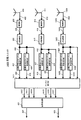•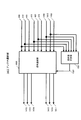•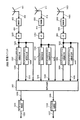•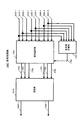•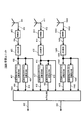•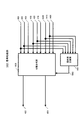•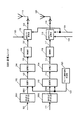•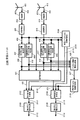•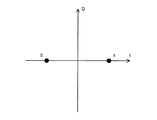•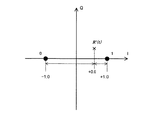•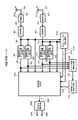•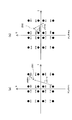•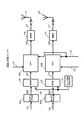•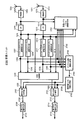•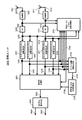•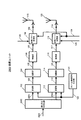•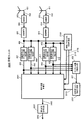•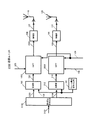•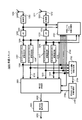•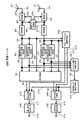•••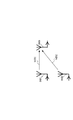•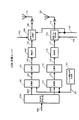•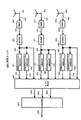••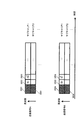•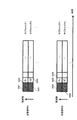•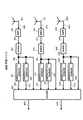•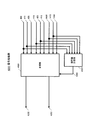•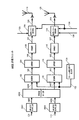•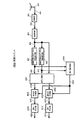•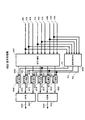•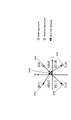••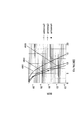•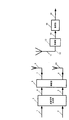## Classifications

• HELECTRICITY
• H04ELECTRIC COMMUNICATION TECHNIQUE
• H04BTRANSMISSION
• H04B7/02Diversity systems; Multi-antenna system, i.e. transmission or reception using multiple antennas
• H04B7/04Diversity systems; Multi-antenna system, i.e. transmission or reception using multiple antennas using two or more spaced independent antennas
• H04B7/0413MIMO systems
• H04B7/0426Power distribution
• H04B7/043Power distribution using best eigenmode, e.g. beam forming or beam steering
• HELECTRICITY
• H04ELECTRIC COMMUNICATION TECHNIQUE
• H04BTRANSMISSION
• H04B17/00Monitoring; Testing
• H04B17/30Monitoring; Testing of propagation channels
• H04B17/309Measuring or estimating channel quality parameters
• HELECTRICITY
• H04ELECTRIC COMMUNICATION TECHNIQUE
• H04BTRANSMISSION
• H04B7/02Diversity systems; Multi-antenna system, i.e. transmission or reception using multiple antennas
• H04B7/04Diversity systems; Multi-antenna system, i.e. transmission or reception using multiple antennas using two or more spaced independent antennas
• H04B7/06Diversity systems; Multi-antenna system, i.e. transmission or reception using multiple antennas using two or more spaced independent antennas at the transmitting station
• H04B7/0613Diversity systems; Multi-antenna system, i.e. transmission or reception using multiple antennas using two or more spaced independent antennas at the transmitting station using simultaneous transmission
• H04B7/0615Diversity systems; Multi-antenna system, i.e. transmission or reception using multiple antennas using two or more spaced independent antennas at the transmitting station using simultaneous transmission of weighted versions of same signal
• H04B7/0619Diversity systems; Multi-antenna system, i.e. transmission or reception using multiple antennas using two or more spaced independent antennas at the transmitting station using simultaneous transmission of weighted versions of same signal using feedback from receiving side
• H04B7/0621Feedback content
• HELECTRICITY
• H04ELECTRIC COMMUNICATION TECHNIQUE
• H04BTRANSMISSION
• H04B17/00Monitoring; Testing
• H04B17/24Monitoring; Testing of receivers with feedback of measurements to the transmitter
• HELECTRICITY
• H04ELECTRIC COMMUNICATION TECHNIQUE
• H04BTRANSMISSION
• H04B7/02Diversity systems; Multi-antenna system, i.e. transmission or reception using multiple antennas
• H04B7/04Diversity systems; Multi-antenna system, i.e. transmission or reception using multiple antennas using two or more spaced independent antennas
• H04B7/08Diversity systems; Multi-antenna system, i.e. transmission or reception using multiple antennas using two or more spaced independent antennas at the receiving station
• H04B7/0837Diversity systems; Multi-antenna system, i.e. transmission or reception using multiple antennas using two or more spaced independent antennas at the receiving station using pre-detection combining
• H04B7/0842Weighted combining
• H04B7/0848Joint weighting
• HELECTRICITY
• H04ELECTRIC COMMUNICATION TECHNIQUE
• H04WWIRELESS COMMUNICATION NETWORKS
• H04W52/00Power management, e.g. TPC [Transmission Power Control], power saving or power classes
• H04W52/04TPC
• H04W52/38TPC being performed in particular situations
• H04W52/42TPC being performed in particular situations in systems with time, space, frequency or polarisation diversity

## Description

The present invention relates to a base station transmission method, a base station transmission apparatus, and a communication terminal , and more particularly to a base station transmission method, a base station transmission apparatus, and a communication terminal applied to a radio communication system using multiple antennas. is there.

2. Description of the Related Art Conventionally, research and development of a wireless communication system using a multi-antenna has been actively performed in order to enable transmission / reception of more data in a limited frequency band. Examples of a wireless communication system using a multi-antenna include a MIMO (Multiple-Input Multiple-Output) system in which a plurality of antennas are provided in both a transmission device and a reception device, and a reception device in which a plurality of antennas are provided in the transmission device. There is a MISO (Multiple-Input Single-Output) system provided with an antenna. Among these, as a technique for improving the reception quality in the MISO system, for example, the invention disclosed in Patent Document 1 is known.

JP 2001-237750 A

By the way, in the wireless communication system using the multi-antenna technology as described above, the modulation signals transmitted from a plurality of antennas are multiplexed on the propagation path and received by the receiving antenna, so that demodulation processing including signal separation processing is enhanced. If it cannot be performed with accuracy, the error rate characteristics of the received data deteriorate, and as a result, the original purpose of improving the data transmission speed cannot be achieved.

In order to improve the separation / demodulation accuracy of each modulation signal, it is conceivable to increase the number of pilot symbols to be inserted into each modulation signal. However, if the number of pilot symbols is increased, the data transmission efficiency decreases accordingly.

The present invention has been made in view of such points, and a base station transmission method, a base station transmission device, and a base station transmission method that can improve demodulation accuracy including separation processing of each modulation signal and improve error rate characteristics of received data, and An object is to provide a communication terminal .

One aspect of a transmitting method for a base station of the present invention is a transmission method of a base station transmitting an independent modulated signals from each of a plurality of antennas, for transmitting different data from each antenna Information indicating the received signal strength when each modulated signal is received from a communication terminal that forms a plurality of modulated signals and receives the modulated signal , and can be effectively used when demodulating each modulated signal receiving feedback information including the information on the effective received signal strength, based on said feedback information, controls the transmission power of the modulated signal transmitted from each antenna independently at each antenna.

One aspect of a transmitting apparatus of the base station of the present invention is a transmission apparatus of the base station transmitting an independent modulated signals from each of a plurality of antennas, for transmitting different data from each antenna Information indicating reception signal strength when each modulation signal is received from a plurality of modulation units that form a plurality of modulation signals, and a communication terminal that receives the modulation signal , and when demodulating each modulation signal A receiving unit that receives feedback information including information on effective received signal strength that can be effectively used , and transmission power that independently controls transmission power of a modulated signal transmitted from each antenna based on the feedback information. A configuration is adopted that includes a control unit and a transmission unit that transmits the modulated signal, the transmission power of which is controlled, from each antenna.

One aspect of the communication terminal of the present invention is a communication terminal that receives a plurality of independent modulated signals transmitted from a plurality of antennas of a base station and demodulates each modulated signal by the plurality of antennas, A reception field strength detection unit that detects a reception field strength of each modulation signal received by a plurality of antennas, a channel fluctuation estimation unit that estimates a channel estimation value of each of the plurality of modulation signals received, and the channel estimation value as elements A demodulator that demodulates the modulated signal using a channel fluctuation estimation matrix, information indicating the received electric field strength detected by the received electric field strength detector, and a channel estimation value estimated by the channel fluctuation estimator A configuration including a feedback signal generation unit that generates a feedback signal to the base station based on the information is adopted.

According to one aspect of the transmission apparatus of the base station of the present invention, the transmission power of the modulated signal transmitted from each antenna is based on information such as the channel fluctuation value of each modulated signal fed back from the communication terminal and the received electric field strength. Te, it controls to each antenna independently. Thereby, since the effective received power of each modulated signal can be changed more accurately, the demodulation accuracy of each modulated signal at the communication terminal can be further improved.

According to the present invention, in a radio communication system using a multi-antenna, demodulation processing and transmission processing are performed in consideration of effective received power of a plurality of modulated signals transmitted from a plurality of antennas. It is possible to realize a base station transmission method, a base station transmission apparatus, and a communication terminal that can improve the accuracy of demodulation including separation processing and improve the error rate characteristics of received data.

The inventors of the present invention do not simply separate and demodulate each modulated signal in a wireless communication system using a multi-antenna, but rather the effective received power of the received modulated signal (that is, the received power obtained by the receiving device). It is considered that the demodulation accuracy of each modulation signal can be improved by performing demodulation processing and transmission processing in consideration of the substantial reception power that can be effectively used when demodulating each modulation signal. It came to.

In the present invention, the eigenvalue of the channel fluctuation matrix is used as an index of effective received power. The channel variation matrix is obtained by associating each antenna reception signal and each modulation signal with a channel variation value as an element in order to separate each modulation signal by the receiving apparatus. That is, it is a matrix having channel fluctuation values as elements. In general, a receiving apparatus used in multi-antenna communication obtains an inverse matrix of a channel fluctuation matrix and separates each modulated signal from the received signal.

In the present invention, the eigenvalue is obtained from the channel variation matrix that is generally used in this way, and this is used as an index of the effective received power. Therefore, the effective received power can be reduced by adding a relatively small amount of calculation and a relatively small configuration. It is made to be able to ask.

In the following embodiments, the following aspects of the present invention will be mainly described.

According to one aspect of the present invention, in a transmission apparatus that transmits a plurality of modulated signals from a plurality of antennas, the transmission power of the plurality of modulated signals to be transmitted is changed independently at each antenna. Further, the transmission power is controlled using the received electric field strength and channel fluctuation estimated by the communication partner. Thereby, the transmission quality of data can be improved. Specifically, since it becomes possible to perform transmission power control of the modulation signal so that the effective reception power is optimized, it is possible to improve the demodulation accuracy of each modulation signal on the reception side.

According to still another aspect of the present invention, a reception device that receives a modulated signal transmitted by the transmission device includes a reception field strength estimation unit that estimates a reception field strength from the reception signal, and transmits the estimated reception field strength information. Feedback was provided to the device. In addition, a channel fluctuation estimation unit for estimating the channel fluctuation of each modulated signal from the received signal is provided, and the estimated channel fluctuation information is fed back to the transmitter. As a result, the transmission apparatus can perform transmission power control of the modulation signal so that the effective reception power on the reception side is actually optimized based on the reception field strength information and channel fluctuation information.

According to still another aspect of the present invention, in a transmission device that transmits a plurality of modulated signals from a plurality of antennas using a multicarrier scheme, the transmission power of the plurality of modulated signals to be transmitted is changed independently at each antenna, and I made it to each carrier independently. Further, the transmission power is controlled by using the received electric field strength for each carrier estimated by the communication partner and the channel fluctuation for each carrier. As a result, transmission power control of the modulated signal can be performed so that the effective reception power is optimized independently for each antenna and independently for each carrier.

According to still another aspect of the present invention, in the receiving device that receives the modulated signal transmitted by the multicarrier transmitting device, a receiving electric field strength estimating unit that estimates a receiving electric field strength for each carrier from the received signal is provided, and for each estimated carrier The received electric field strength information is fed back to the multicarrier transmission apparatus. In addition, a channel fluctuation estimation unit for estimating the channel fluctuation for each carrier from the received signal is provided, and the estimated channel fluctuation information for each carrier is fed back to the multicarrier transmission apparatus. As a result, the multicarrier transmission apparatus performs transmission power control of the modulated signal for each carrier so that the effective reception power on the reception side is actually optimized based on the reception field strength information and channel fluctuation information for each carrier. Will be able to.

According to still another aspect of the present invention, in a receiving apparatus that receives a plurality of modulated signals transmitted from a plurality of antennas by a plurality of receiving antennas that are larger than transmitting antennas, a combination of a plurality of antenna received signals is created for each combination. A channel variation matrix is formed, an eigenvalue of the channel variation matrix for each combination is calculated, and an antenna reception signal having a maximum minimum eigenvalue power is selected to perform demodulation processing. As a result, each modulation signal can be demodulated using a combination of antenna reception signals that maximizes the effective reception power of the modulation signal. Compared to the case where each modulation signal is demodulated using all antenna reception signals. Thus, the demodulation accuracy of the modulation signal can be improved.

According to another aspect of the present invention, in a receiving apparatus that receives a plurality of modulated signals transmitted from a plurality of antennas using a plurality of receiving antennas that are greater than the number of transmitting antennas, a combination of a plurality of antenna received signals is created. A channel variation matrix is formed at e, and eigenvalues of the channel variation matrix for each combination are calculated. Then, each modulation signal is separated using the antenna reception signal of each combination and the channel variation matrix corresponding to the combination, and the eigenvalue of the channel variation matrix used for separation of the modulation signal separated by each combination is used. Weighted composition. As a result, each modulation signal can be weighted and synthesized according to the effective received power of the modulation signal, so that the demodulation accuracy of the modulation signal can be improved.

According to still another aspect of the present invention, an eigenvalue of a channel variation matrix is obtained in a receiving apparatus that receives a plurality of modulated signals that have been error correction encoded and transmitted from a plurality of antennas, and is obtained from the eigenvalue and a received quadrature baseband signal. A soft decision value calculation unit for obtaining a soft decision value is provided.

According to another aspect of the present invention, an effective reception level is obtained from a reception level and an eigenvalue of a channel variation matrix in a receiving apparatus that receives a plurality of modulated signals that have been error correction encoded and transmitted from a plurality of antennas. A soft decision value calculation unit for obtaining a soft decision value from the reception level and the reception quadrature baseband signal is provided.

Thus, by obtaining the soft decision value by weighting with the effective reception level, it is possible to give the soft decision value an appropriate likelihood, and the demodulation accuracy of the modulation signal can be improved.

In another aspect of the present invention, when performing demodulation processing using eigenvalues of the channel variation matrix, control of the signal level of the received signal received by each antenna is commonly performed for each antenna. . Thereby, since the eigenvalue can be obtained more accurately, demodulation processing can be performed based on the eigenvalue that more accurately reflects the effective received power, so that the demodulation accuracy of each modulation signal can be further improved.

Embodiments of the present invention will be described below with reference to the accompanying drawings.

(Embodiment 1)
In the present embodiment, a description will be given of a transmission apparatus that independently changes the transmission power of a modulated signal transmitted from each antenna.

The transmission power changing unit 108 receives the modulated signal 107, the coefficient 125 obtained from the received power, and the coefficient 124 obtained from the eigenvalue, and obtains the transmission signal 109 by multiplying the modulated signal 107 by the coefficients 125 and 124 and outputs this. To do. Thereby, the transmission power of the modulated signal 107 is determined based on the reception power and the eigenvalue. The transmission signal 109 is output from the antenna 110 as a radio wave.

The transmission power changing unit 118 receives the modulation signal 117, the coefficient 126 obtained from the received power, and the coefficient 124 obtained from the eigenvalue, and obtains the transmission signal 119 by multiplying the modulation signal 117 by the coefficients 126 and 124, and outputs this. To do. Thereby, the transmission power of the modulated signal 117 is determined based on the reception power and the eigenvalue. The transmission signal 119 is output from the antenna 120 as a radio wave.

As described above, in the transmission unit 100 provided in the transmission apparatus of the present embodiment, the transmission power of the modulated signal transmitted from each antenna 110, 120 can be changed independently.

The data separation unit 207 receives the received digital signal 206, separates the received digital signal 206 into data 208, field strength estimation information 209, and channel fluctuation estimation information 210, and outputs them.

Coefficient calculation unit 211 based on reception power receives electric field strength estimation information 209 as an input, and based on this electric field strength estimation information 209, calculates coefficients 125 and 126 used by transmission power changing units 108 and 118 of transmission unit 100 to transmit power. The data is sent to the changing units 108 and 118. Details of how to obtain the coefficients 125 and 126 will be described later.

The coefficient calculation unit 214 based on the eigenvalue receives the channel fluctuation estimation information 210 as an input, calculates the coefficient 124 used in the transmission power changing units 108 and 118 of the transmission unit 100 based on the channel fluctuation estimation information 210, and transmits the transmission power changing unit 108. , 118. Details of how to obtain the coefficient 124 will be described later.

FIG. 3 shows an example of a frame structure on the time axis of each transmission signal 109 (spread signal A) and 119 (spread signal B) transmitted from each antenna 110 and 120 of the transmission unit 100. Spread signal A shown in FIG. 3A and spread signal B shown in FIG. 3B are transmitted simultaneously from antennas 110 and 120. The channel estimation symbol 301 of the spread signal A and the channel estimation symbol 301 of the spread signal B are, for example, codes orthogonal to each other. As a result, the receiving unit of the terminal can estimate the channel fluctuations of the signals transmitted from the antennas 110 and 120 based on the channel estimation symbols 301 included in the spread signals A and B, respectively.

The signal processing unit 421 receives the received quadrature baseband signals 406 and 416, the channel fluctuation estimation signals 408 and 418 of the spread signal A, and the channel fluctuation estimation signals 410 and 420 of the spread signal B, and receives the channel fluctuation estimation values 408, 410, and 418. , 420 are used to calculate the reception orthogonal baseband signal 422 of the spread signal A and the reception orthogonal baseband signal 423 of the spread signal B. Details of this channel variation matrix will be described later.

Reception field strength estimation section 424 receives reception quadrature baseband signals 406 and 416, obtains reception field strength of these signals, and outputs reception field strength estimation information 425. In this embodiment, the received electric field strength is obtained from the received quadrature baseband signal, but is not limited to this, and may be obtained from the received signal. The received electric field strength may be obtained separately for the spread signal A and the spread signal B, or the received electric field strength of the composite wave may be obtained.

The channel fluctuation information generation unit 426 receives the channel fluctuation estimation signals 408 and 418 of the spread signal A and the channel fluctuation estimation signals 410 and 420 of the spread signal B, and forms and outputs the channel fluctuation estimation information 427.

FIG. 5 shows an example of the configuration of the transmission unit of the reception apparatus in this embodiment. The transmission unit 500 is provided in the same communication terminal as the reception unit 400. The information generation unit 504 of the transmission unit 500 receives the data 501, the received electric field strength estimation information 425, and the channel fluctuation estimation information 427, and arranges them in a predetermined order to output a transmission digital signal 505. Modulation signal generation section 506 receives transmission digital signal 505 as input, and performs modulation processing on transmission digital signal 505 to form modulation signal 507 and output it. The radio unit 508 receives the modulated signal 507 as input, and forms and outputs a transmission signal 509 by performing predetermined radio processing such as digital-analog conversion processing and up-conversion on the modulated signal 507. A transmission signal 509 is output from the antenna 510 as a radio wave.

FIG. 6 shows an example of a frame configuration of a transmission signal transmitted from the transmission unit 500. In the figure, 601 is a channel fluctuation estimation information symbol, 602 is a field strength estimation information symbol, and 603 is a data symbol.

FIG. 7 shows an example of the relationship between the transmission signal and the reception signal. Modulated signal Ta (t) transmitted from transmitting antenna 110 is received by antennas 401 and 402 after receiving channel fluctuations h11 (t) and h12 (t). The modulated signal Tb (t) transmitted from the transmission antenna 120 is received by the antennas 401 and 402 after receiving the channel fluctuations h21 (t) and h22 (t).

Next, operations of the transmission apparatus and the reception apparatus in the present embodiment will be described in detail with reference to FIGS.

First, the transmission operation of the base station (transmission apparatus) will be described. An important operation in the transmission unit 100 of the base station shown in FIG. 1 is that the transmission power of the modulated signal transmitted from each antenna 110 and 120 is independently controlled by each antenna 110 and 120. For this reason, in the transmission unit 100, the transmission power changing sections 108 and 118 multiply the transmission signal by a coefficient.

Here, the operation of the transmission power changing unit 108 will be described in detail. Assuming that the value of the multiplication coefficient 125 obtained from the received power is Ca, the modulation signal 107 is Xa (t), and the coefficient 124 obtained from the eigenvalue is D, the transmission power changing unit 108 represents the transmission signal 109 as expressed by the following equation. The transmission power Xa ′ (t) is controlled.

Similarly, transmission power changing section 118 performs transmission as represented by the following equation, where Cb is the value of multiplication coefficient 126 obtained from the received power, Xb (t) is the modulation signal 117, and D is the coefficient 124 obtained from the eigenvalue. The transmission power Xb ′ (t) of the signal 119 is controlled.

Thus, the reception quality can be improved by performing transmission power control independently for each transmission antenna. In addition, since the value D of the coefficient 124 obtained from the eigenvalue is multiplied in common by the transmission power changing units 108 and 118 of all the transmitting antennas, the reception quality can be further effectively improved. This is because the coefficient obtained from the eigenvalue corresponds to the effective received electric field strength of the receiving terminal (the actual received electric field strength that can be effectively used among the received electric field strengths obtained at the terminal).

In addition, since the coefficient obtained from the reception power is multiplied independently by the transmission power changing sections 108 and 118 of the respective transmission antennas, the reception quality can be improved more effectively. This is because the coefficient obtained from the reception power corresponds to transmission power control for improving the reception electric field strength of each modulation signal at the antenna of the reception terminal.

Next, the receiving operation of the base station (transmitting device) will be described. As shown in FIG. 7, t is time, the modulation signal from the antenna 110 is Ta (t), the modulation signal from the antenna 120 is Tb (t), the reception signal of the antenna 401 is R1 (t), and the antenna 402 When the received signal is R2 (t) and the channel fluctuation is h11 (t), h12 (t), h21 (t), and h22 (t), the following relationship is established. That is, each antenna reception signal R1 (t), R2 (t) and each modulation signal Ta (t), Tb (t) are converted into channel fluctuation values h11 (t), h12 (t), h21 (t), h22 ( It can be related by a channel variation matrix with t) as an element.

The coefficient calculation unit 211 based on reception power provided in the reception unit 200 of the base station (transmission apparatus) in FIG. 2 receives the field strength estimation information 209 received from the terminal, that is, the reception field strengths of R1 (t) and R2 (t). The coefficients 125 and 126 are determined using the channel fluctuation estimation information 210, that is, h11 (t), h12 (t), h21 (t), and h22 (t).

For example, the coefficient 125 is obtained from the estimated values of h11 (t) and h21 (t). This is because h11 (t) and h21 (t) are fluctuation values determined by the transmission power of the signal output from the antenna 110 in FIG. Similarly, the coefficient 126 is obtained from the estimated values of h12 (t) and h22 (t). This is because h12 (t) and h22 (t) are fluctuation values determined by the transmission power of the signal output from the antenna 120 of FIG.

That is, the received electric field strengths of R1 (t) and R2 (t) are both the electric field strengths of the signal obtained by combining the signal from the antenna 110 and the signal from the antenna 120. Is insufficient to properly adjust the signal power from each antenna. Therefore, in this embodiment, in addition to the received electric field strength, each antenna 110 is used by using channel fluctuation values h11 (t), h21 (t), h12 (t), and h22 (t) at the time of receiving each transmission signal. , 120 to determine the coefficients 125 and 126 for controlling the signal power to be transmitted. Thereby, the power at the time of reception of each signal transmitted from each antenna 110 and 120 can be made appropriate.

More specifically, when the received electric field strength is small, the values of the coefficients 125 and 126 are increased so that the transmission power is naturally increased. Furthermore, as the channel fluctuation values h11 (t) and h21 (t) are smaller, the value of the coefficient 125 used in the antenna 110 is increased. Similarly, as the channel fluctuation values h12 (t) and h22 (t) are smaller, the value of the coefficient 126 used in the antenna 120 is increased.

The coefficient calculation unit 214 based on eigenvalues is an eigenvalue of the channel fluctuation matrix of Equation (3) whose elements are estimated values of channel fluctuations h11 (t), h12 (t), h21 (t), and h22 (t) received from the terminal. , And the coefficient 124 is obtained based on the smallest power among the eigenvalue powers.

Here, the calculation method for obtaining the eigenvalue includes, for example, the Jacobi method, Givens method, Housefolde method, QR method, QL method, QL method with implicit shift, and inverse iteration method. In the present invention, any calculation method is used. Also good. The power of the eigenvalue is a value represented by a 2 + b 2 when the eigenvalue is represented as a + bj (a, b: real number, j: imaginary number). The same applies to other embodiments described later.

Next, the receiving operation of the communication terminal (receiving device) will be described. The channel fluctuation estimation unit 407 of the spread signal A of the reception unit 400 of FIG. 4 determines the channel fluctuation of the spread signal A, that is, h11 (t) in the expression (3) from the channel estimation symbol 301 of the spread signal A shown in FIG. And the estimation result is output as the channel fluctuation estimation signal 408 of the spread signal A. The channel fluctuation estimation unit 409 of the spread signal B estimates the channel fluctuation of the spread signal B, that is, h12 (t) in the equation (3), from the channel estimation symbol 301 of the spread signal B shown in FIG. The channel fluctuation estimation signal 410 of the spread signal B is output.

The channel fluctuation estimation unit 417 of the spread signal A estimates the channel fluctuation of the spread signal A, that is, h21 (t) in the expression (3), from the channel estimation symbol 301 of the spread signal A shown in FIG. The channel fluctuation estimation signal 418 of the spread signal A is output. The channel fluctuation estimation unit 419 of the spread signal B estimates the channel fluctuation of the spread signal B, that is, h22 (t) in the equation (3) from the channel estimation symbol 301 of the spread signal B shown in FIG. The channel fluctuation estimation signal 420 of the spread signal B is output.

The signal processing unit 421 obtains the reception orthogonal baseband signals 422 and 423 of the spread signals A and B by performing an inverse matrix operation by multiplying both sides by the inverse matrix of the channel variation matrix in the expression (3). As a result, the reception quadrature baseband signal 422 and the reception quadrature baseband signal 423 are separated. The channel fluctuation information generation unit 426 uses the estimated channel fluctuations h11 (t), h12 (t), and h21 (as channel fluctuation estimation signals 408 and 418 of the spread signal A and channel fluctuation estimation signals 410 and 420 of the spread signal B. t) and h22 (t) are input and output as channel fluctuation estimation information 427.

Thus, according to the above configuration, in a transmission apparatus that performs multi-antenna transmission, channel fluctuation values h11 (t), h12 (t), h21 (t), h22 at the time of receiving modulated signals transmitted from the antennas 110 and 120 are received. (T) is received from the communication partner station, and the transmission power of the modulated signal transmitted from each antenna 110, 120 based on the channel fluctuation values h11 (t), h12 (t), h21 (t), h22 (t) Since the antennas 110 and 120 are independently controlled, the reception electric field strength at the time of receiving each modulation signal can be made appropriate, and the reception quality of each modulation signal can be improved.

In addition, the transmission power is controlled by taking into account the eigenvalues of the channel variation matrix having the channel variation values h11 (t), h12 (t), h21 (t), and h22 (t) as elements. Since the reception electric field strength can be increased, the reception quality of each modulated signal can be further improved.

In the above-described embodiment, the case where the base station, that is, the transmission side, determines the coefficients 124, 125, and 126 for controlling the transmission power of the antennas 110 and 120 has been described. However, the present invention is not limited to this. Coefficients 124, 125, and 126 may be determined at the terminal, that is, the reception side, and the determined coefficients may be fed back to the transmission side. The same applies to other embodiments described below.

In the above-described embodiment, the case where the number of antennas is two and the number of modulated signals to be multiplexed is two has been described. However, the present invention is not limited to this, and in short, the modulation signals differ from each antenna using a plurality of antennas. It can be widely applied when transmitting. Further, for example, one antenna (for example, the antenna 110) that transmits each modulation signal, such as an adaptive array antenna, may be configured by a plurality of antennas. The same applies to other embodiments described below.

In the above-described embodiment, the reception electric field strength is described. However, the reception electric field strength is replaced with a reception level, reception intensity, reception power, reception amplitude, carrier power versus noise power, or the like. Can do. The same applies to other embodiments described below.

In the embodiment described above, a symbol to be transmitted to estimate channel fluctuation is called a channel estimation symbol 301 (FIG. 3). However, the channel estimation symbol 301 is a pilot symbol, preamble, control symbol, known symbol, or unique word. You may call it, and you may call it with another name. Moreover, the channel fluctuation estimation information symbol 601 and the electric field strength estimation information symbol 602 in FIG. 6 may be called control symbols or may be called with other names. That is, even if these symbols are used, the present invention can be implemented in the same manner as in the above embodiment. The same applies to other embodiments described below.

In the above-described embodiment, the spread spectrum communication method has been described as an example. However, the present invention is not limited to this. For example, the present invention can be similarly applied to a single carrier method and an OFDM method that do not have a spreading unit. In the case of the single carrier method, the diffusion units 104 and 114 (FIG. 1) and the despreading units 405 and 415 (FIG. 4) are not provided. The same applies to all of the following embodiments that will be described by taking the spread spectrum method as an example. The case where the present invention is applied to the OFDM system will be described in detail in Embodiment 2.

Furthermore, the configurations of the transmission device and the reception device of the present invention are not limited to the configurations of FIGS. 1, 2, 4 and 5. For example, in the above-described embodiment, the transmission power changing units 108 and 118 are provided, and the transmission power changing unit uses the antennas 110 and 120 based on the coefficients 124 obtained from the eigenvalues and the coefficients 125 and 126 obtained from the received power. Although the case where the transmission power of the modulation signal to be transmitted is controlled independently by each antenna 110, 120 has been described, the point is that the modulation signal at each antenna may be controlled independently, and the configuration is shown in FIG. It is not limited to what is shown.

FIG. 8 shows another configuration example of the transmission unit of the base station in the present embodiment. In FIG. 8, components that operate in the same manner as the transmission unit 100 of FIG. The transmission unit 700 in FIG. 8 differs from the transmission unit 100 in FIG. 1 in that the transmission unit 100 in FIG. 1 controls the power of the modulated signal transmitted from each antenna by the transmission power changing units 108 and 118. In other words, the power of the modulated signal transmitted from each antenna is controlled by the spreading sections 701 and 702.

Specifically, the spreading unit 701 receives the transmission quadrature baseband signal 103, the coefficient 125 obtained from the received power, and the coefficient 124 obtained from the eigenvalue, and outputs the spread signal 105 having the power corresponding to these coefficients 125 and 124. To do. Similarly, the spreading unit 702 receives the transmission quadrature baseband signal 113, the coefficient 126 obtained from the received power, and the coefficient 124 obtained from the eigenvalue, and outputs a spread signal 115 having a power corresponding to these coefficients 126 and 124.

The coefficient multiplication function unit 810 receives the channel X spread signal 805, the channel Y spread signal 806, the channel Z spread signal 807, the coefficient 125 (126) obtained from the received power, and the coefficient 124 obtained from the eigenvalue. By multiplying the coefficients according to the coefficients 125 (126) and 124, a channel X spread signal 811 after coefficient multiplication, a channel Y spread signal 812 after coefficient multiplication, and a channel Z spread signal 813 after coefficient multiplication are formed. And output.

Here, the coefficient 125 (126) obtained from the received power and the coefficient 124 obtained from the eigenvalue multiplied by the spread signal 805 of the channel X are based on the received electric field strength estimation information and the channel fluctuation estimation information transmitted from the terminal X. It is what was sought. Further, the coefficient 125 (126) obtained from the received power and the coefficient 124 obtained from the eigenvalue multiplied by the spread signal 806 of the channel Y are obtained based on the received electric field strength estimation information and the channel fluctuation estimation information sent from the terminal Y. It is what was done. The coefficient 125 (126) obtained from the received power and the coefficient 124 obtained from the eigenvalue multiplied by the spread signal 807 of the channel Z are obtained based on the received electric field strength estimation information and the channel fluctuation estimation information sent from the terminal Z. It is what was done.

The addition function unit 814 adds the spread signal 811 of the channel X after the coefficient multiplication, the spread signal 812 of the channel Y after the coefficient multiplication, and the spread signal 813 of the channel Z after the coefficient multiplication, and outputs the result as the spread signal 105 (115). To do.

As described above, the transmission unit 700 is configured to simultaneously generate transmission signals addressed to a plurality of terminals. At this time, the transmission unit 700 receives the received electric field strength estimation information and the channel fluctuation estimation information from each terminal, obtains a coefficient obtained from the received power for each terminal, a coefficient obtained from the eigenvalue, and these coefficients that are different for each terminal. Is multiplied by the spread modulation signal addressed to each terminal, so that the transmission power can be controlled independently of each antenna and independently of the modulation signal addressed to each terminal. As a result, when simultaneously transmitting modulated signals addressed to a plurality of terminals from a plurality of antennas, it is possible to optimize the effective received power in all of the plurality of terminals and improve the reception quality of all of the plurality of terminals without reducing the transmission rate. become.

Thus, according to the present embodiment, information serving as an index of effective received power, such as channel fluctuation information and received electric field strength information, is received as feedback information from the receiving apparatus, and transmission of modulated signals transmitted from each antenna based on this information By changing the power independently for each antenna, the effective reception power of the modulated signal transmitted from each antenna can be increased, and a transmission apparatus capable of improving the reception quality of the modulated signal can be realized.

(Embodiment 2)
In the present embodiment, a description will be given of a transmission apparatus that changes the transmission power of a modulated signal transmitted from each antenna independently for each antenna and independently for each carrier.

FIG. 10 shows an example of the configuration of the transmission unit of the transmission apparatus in this embodiment. The transmission unit 1000 is provided in a base station, for example. The reception unit of the base station is configured as shown in FIG. 2, for example, and the transmission unit of the terminal that communicates with the base station is configured as shown in FIG. 5, for example, and is transmitted from the transmission unit of the terminal. The frame structure of the signal is as shown in FIG. 6, for example, and since these have already been described in the first embodiment, description thereof will be omitted.

The transmission unit 1000 inputs the transmission digital signal 101 and the timing signal 122 to the modulation unit 102, subjects the transmission digital signal 101 to quadrature modulation processing such as QPSK and 16QAM, and the frame configuration according to the timing signal 122 (FIG. 11 (A)), the transmission quadrature baseband signal 103 is formed and output. The IDFT 1001 receives the transmission quadrature baseband signal 103, the coefficient 125 obtained from the received power, and the coefficient 124 obtained from the eigenvalue, changes the transmission power based on the coefficients 125 and 124, performs inverse Fourier transform, and performs inverse Fourier transform. The signal 1002 is output.

Similarly, the transmission unit 1000 inputs the transmission digital signal 111 and the timing signal 122 to the modulation unit 112, performs quadrature modulation processing such as QPSK and 16QAM on the transmission digital signal 111, and a frame according to the timing signal 122. The transmission quadrature baseband signal 113 is formed and output by adopting the configuration (FIG. 11B). The IDFT 1003 receives the transmission quadrature baseband signal 113, the coefficient 126 obtained from the received power, and the coefficient 124 obtained from the eigenvalue, changes the transmission power based on the coefficients 126 and 124, performs inverse Fourier transform, and performs inverse Fourier transform. The later signal 1004 is output.

FIG. 11 shows a frame configuration example of a modulated signal transmitted from the transmission unit 1000. FIG. 11A shows a frame configuration of a signal (channel A) transmitted from the antenna 110, and FIG. 11B shows a frame configuration of a signal (channel B) transmitted from the antenna 120. In this example, the estimation symbols 1101 are arranged and transmitted on all subcarriers at a specific time 1, and the information symbols 1102 are transmitted at other times 2 to 9.

Channel A channel fluctuation estimation section 1207 receives Fourier-transformed signal 1206 as input, estimates channel fluctuation of channel A signal (OFDM signal transmitted from antenna 110) based on channel A channel estimation symbol, and performs channel fluctuation. An estimated group signal 1208 is output. Thereby, the channel fluctuation between the antenna 110 and the antenna 1201 is estimated. Channel B channel fluctuation estimation section 1209 receives Fourier transformed signal 1206 as input, estimates channel fluctuation of channel B signal (OFDM signal transmitted from antenna 120) based on channel B channel estimation symbols, and performs channel fluctuation. The estimated group signal 1210 is output. Thereby, the channel fluctuation between the antenna 120 and the antenna 1201 is estimated.

Channel A channel fluctuation estimation section 1217 receives signal 1216 after Fourier transform as input, estimates the channel fluctuation of channel A signal (OFDM signal transmitted from antenna 110) based on channel A channel estimation symbols, and changes the channel fluctuation. The estimated group signal 1218 is output. Thereby, the channel fluctuation between the antenna 110 and the antenna 1211 is estimated. Channel B channel fluctuation estimation section 1219 receives Fourier transformed signal 1216 as input, estimates the channel fluctuation of channel B signal (OFDM signal transmitted from antenna 120) based on channel B channel estimation symbols, and changes the channel fluctuation. An estimated group signal 1220 is output. Thereby, the channel fluctuation between the antenna 120 and the antenna 1211 is estimated.

The signal processor 1221 receives the Fourier transformed signals 1206 and 1216, channel A channel fluctuation estimation signals 1208 and 1218, and channel B channel fluctuation estimation signals 1210 and 1220 as input, and channel fluctuation estimation values 1208, 1218, 1210 and 1220. The channel A reception orthogonal baseband signal group 1222 and the channel B reception orthogonal baseband signal group 1223 are output by performing an operation using the inverse matrix of the channel variation matrix having the elements as.

The channel A demodulation unit 1224 receives the channel A reception quadrature baseband signal group 1222 and performs a demodulation process corresponding to the modulation unit 102 of the transmission unit 1000 (FIG. 10) on the received signal to receive the digital signal 1225. Is output. Channel B demodulator 1226 receives channel B received quadrature baseband signal group 1223 as input, and performs a demodulation process corresponding to modulator 112 of transmitter unit 1000 to form a received digital signal 1227. Output.

Received electric field strength estimating section 1228 receives signals 1206 and 1216 after Fourier transformation, obtains received electric field strengths of these signals, and outputs received electric field strength estimation information 1229.

Channel fluctuation estimation section 1230 receives channel fluctuation estimation signal groups 1208 and 1218 for channel A and channel fluctuation estimation signal groups 1210 and 1220 for channel B, and forms and outputs channel fluctuation estimation information 1231.

FIG. 13 shows a configuration example of IDFTs 1001 and 1003 provided in the transmission unit 1000 of FIG. Here, since the IDFT 1001 and the IDFT 1003 have the same configuration, the IDFT 1001 will be described.

The IDFT 1001 has a transmission power changing unit 1307. The transmission power changing unit 1307 is based on the transmission orthogonal baseband signal 1301 of carrier 1, the transmission orthogonal baseband signal 1302 of carrier 2, the transmission orthogonal baseband signal 1303 of carrier 3, the transmission orthogonal baseband signal 1304 of carrier 4, and the reception power. By using the obtained coefficient 125 and the coefficient 124 obtained from the eigenvalue as inputs and multiplying the transmission orthogonal baseband signals 1301 to 1304 of the respective carriers by the coefficients 125 and 124, the transmission orthogonal baseband signal 1308 of the carrier 1 after the coefficient multiplication, The transmission orthogonal baseband signal 1309 of carrier 2 after coefficient multiplication, the transmission orthogonal baseband signal 1310 of carrier 3 after coefficient multiplication, and the transmission orthogonal baseband signal 1311 of carrier 4 after coefficient multiplication are obtained and output.

Here, the coefficient 125 obtained from the received power and the coefficient 124 obtained from the eigenvalue in this embodiment are obtained for each carrier. The transmission power changing unit 1307 multiplies the transmission orthogonal baseband signals of the carriers corresponding to each other and the coefficients 125 and 124 to change the transmission power for each carrier.

The inverse Fourier transform unit (IDFT unit) 1312 includes a transmission orthogonal baseband signal 1308 of carrier 1 after coefficient multiplication, a transmission orthogonal baseband signal 1309 of carrier 2 after coefficient multiplication, and a transmission orthogonal baseband of carrier 3 after coefficient multiplication. The signal 1310 and the transmission quadrature baseband signal 1311 of the carrier 4 after the coefficient multiplication are input, and inverse Fourier transform processing is performed on these signals to obtain a signal 1313 after inverse Fourier transform and output it.

Next, operations of the transmission device and the reception device in the present embodiment will be described in detail. Here, in order to simplify the description, the drawings (FIGS. 2 and 6) used in Embodiment 1 are used.

First, the operation of the base station (transmitting device) will be described. An important operation in the transmission unit 1000 of the base station shown in FIG. 10 is that the transmission power of the OFDM signal transmitted from each antenna 110, 120 is controlled independently by each antenna 110, 120. In addition, the transmission power is controlled for each carrier. Therefore, the transmission unit 1000 multiplies a coefficient to change the transmission power of the transmission orthogonal baseband signals 103 and 113 in the IDFTs 1001 and 1003.

The detailed operation will be described with reference to FIG. FIG. 13 shows a detailed configuration of the IDFTs 1001 and 1003 in FIG. The transmission orthogonal baseband groups 103 and 113 of FIG. 10 are the transmission orthogonal baseband signal 1301 of carrier 1, the transmission orthogonal baseband signal 1302 of carrier 2, the transmission orthogonal baseband signal 1303 of carrier 3, and the transmission of carrier 4. This corresponds to the orthogonal baseband signal 1304, and there is an orthogonal baseband signal for each subcarrier.

The transmission power changing unit 1307 multiplies the transmission orthogonal baseband signals of the carriers corresponding to each other and the coefficients 125 and 124 to change the transmission power for each carrier. That is, the coefficient 125 and the eigenvalue 126 obtained from the received power are composed of coefficients for each carrier. The coefficient multiplication method by transmission power changing section 1307 is basically the same as that described in Embodiment 1 except that the coefficient is multiplied for each carrier.

Next, the receiving operation of the base station (transmitting device) will be described. In the case of this embodiment, the receiving unit 200 of FIG. 2 receives the electric field strength estimation information 209 for each carrier from the communication terminal (receiving device) and also receives the channel estimation information 210 for each carrier. Then, coefficients 125 and 126 for each carrier are obtained by a coefficient calculation unit 211 based on received power, and a coefficient 124 for each carrier is obtained by a coefficient calculation unit 214 based on eigenvalues. In this manner, the coefficients 125, 126, and 124 for each carrier are obtained based on the field strength estimation information 209 and the channel fluctuation estimation information 210 for each carrier sent from the communication terminal (receiving device). Note that the coefficient calculation method by the coefficient calculation unit 211 based on received power and the coefficient calculation unit 214 based on eigenvalues is basically the same as that described in Embodiment 1, except that the coefficient is calculated for each carrier.

Next, the receiving operation of the communication terminal (receiving device) will be described. Signals 1206 and 1216 after Fourier transform output from Fourier transform sections (dft) 1205 and 1215 of reception unit 1200 in FIG. 12 are configured by signals for each carrier.

Channel A channel fluctuation estimation section 1207 detects estimation symbol 1101 in FIG. 11A and estimates channel fluctuation for each carrier. That is, h11 (t) in equation (3) is estimated for each carrier and output as a channel fluctuation estimation signal group 1208 for channel A. Channel B channel fluctuation estimation section 1209 detects estimation symbol 1101 in FIG. 11B and estimates channel fluctuation for each carrier. That is, h12 (t) in equation (3) is estimated for each carrier, and is output as a channel fluctuation estimation signal group 1210 for channel B.

Channel A channel fluctuation estimation section 1217 detects estimation symbol 1101 in FIG. 11A and estimates channel fluctuation for each carrier. That is, h21 (t) in equation (3) is estimated for each carrier and output as channel fluctuation estimation signal group 1218 for channel A. Channel fluctuation channel estimation section 1219 of channel B detects estimation symbol 1101 in FIG. 11B and estimates channel fluctuation for each carrier. That is, h22 (t) in equation (3) is estimated for each carrier and output as channel fluctuation estimation signal group 1219 for channel B.

Received electric field strength estimating section 1228 receives signals 1206 and 1216 after Fourier transform as input, obtains the received electric field strength for each carrier, and outputs it as received electric field strength estimated signal 1229.

Channel fluctuation estimation section 1230 receives channel fluctuation estimation signal groups 1208 and 1218 for channel A and channel fluctuation estimation signal groups 1210 and 1220 for channel B, generates channel fluctuation estimation information for each carrier, and provides channel fluctuation estimation information. 1231 is output.

The reception field strength estimation information for each carrier and the channel fluctuation estimation information for each carrier formed in this way are sent as feedback information to the base station by the transmission unit 500 as shown in FIG. 5 corresponds to 1229 in FIG. 12, and channel fluctuation estimation information 427 in FIG. 5 corresponds to 1231 in FIG.

Thus, according to the present embodiment, when a multicarrier signal is transmitted from a plurality of antennas, information that serves as an index of effective received power, such as channel fluctuation information for each carrier and received field strength information for each carrier, is fed back from the receiving device. By changing the transmission power of the multicarrier signal transmitted from each antenna based on this information independently for each antenna and each carrier independently, the effective multicarrier signal transmitted from each antenna is changed. The reception power can be increased for each carrier, and a transmission apparatus that can improve the error rate characteristics of a multicarrier signal over all carriers can be realized.

In this embodiment, the case where the IDFTs 1001 and 1003 change the transmission power for each carrier multicarrier has been described. However, the transmission power may not be changed by the IDFTs 1001 and 1003. For example, the modulation units 102 and 112, You may make it change with the radio | wireless parts 106 and 116. FIG.

In this embodiment, the OFDM method has been described as an example. However, a method and a method combining OFDM processing and spreading processing (for example, OFDM-CDMA method) can be similarly implemented.

(Embodiment 3)
In the present embodiment, in a receiving apparatus that receives a plurality of modulated signals transmitted from a plurality of antennas using a plurality of antennas, the receiving antenna is selected, and only the received signals from the selected receiving antennas are used. A receiving apparatus that performs demodulation will be described.

Specifically, a combination of a plurality of antenna reception signals is created, a channel variation matrix is formed for each combination, an eigenvalue of the channel variation matrix for each combination is calculated, and an antenna reception signal of a combination having the largest eigenvalue minimum power Is selected to perform demodulation processing.

FIG. 14 illustrates an example of a configuration of a reception unit of the reception device in this embodiment. 14, parts corresponding to those in FIG. 4 are given the same reference numerals, and descriptions thereof are omitted. The receiving unit 1400 is provided in a communication terminal, for example. Here, the transmission unit of the base station that communicates with the communication terminal provided with the reception unit 1400 is configured as shown in FIG. 1, for example, and the signal transmitted from the base station is configured as shown in FIG. It shall be.

The reception unit 1400 includes three antennas 401, 411, and 1401, and receives the two modulated signals (spread signal A and spread signal B) transmitted from the transmission unit 100 of FIG. It is supposed to be.

The antenna selection unit 1411 receives the channel fluctuation estimation signals 408, 418, and 1408 of the spread signal A, the channel fluctuation estimation signals 410, 420, and 1410 of the spread signal B, and the received orthogonal baseband signals 406, 416, and 1406 after despreading. Then, an optimum combination of antenna reception signals to be demodulated is selected. The selection method will be described later. The antenna selection unit 1411 outputs channel fluctuation estimation signals 1412 and 1415 of the selected spread signal A, channel fluctuation estimation signals 1413 and 1416 of the selected spread signal B, and selected reception orthogonal baseband signals 1414 and 1417 after despreading. To do.

FIG. 15 shows a configuration example of the antenna selection unit 1411. The antenna selection unit 1411 includes an eigenvalue calculation unit 1501 and a signal selection unit 1503. Eigenvalue calculation section 1501 receives spread signal A channel fluctuation estimation signals 408, 418, 1408 and spread signal B channel fluctuation estimation signals 410, 420, 1410. That is, in this embodiment, since three antennas are provided, three channel fluctuation values are input. Then, two channel combinations are created from the three channel variation values (in this embodiment, there are three combinations), a channel variation matrix is created for each combination, and eigenvalues of each channel variation matrix are generated. Calculate Based on the calculation result of the eigenvalue, two systems of signals for performing the inverse matrix calculation are selected, and a control signal 1502 indicating which two systems are selected is output.

The signal selection unit 1503 controls the spread signal A channel fluctuation estimation signals 408, 418, and 1408, the spread signal B channel fluctuation estimation signals 410, 420, and 1410, the received orthogonal baseband signals 406, 416, and 1406 after despreading, and the control. Based on the control signal 1502, two systems of signals (signals of two antennas) are input to the channel fluctuation estimation signals 1412 and 1415 of the selected spread signal A and the channel fluctuation estimation of the selected spread signal B. Signals 1413 and 1416 and the selected despread received orthogonal baseband signals 1414 and 1417 are output.

Next, operations of the transmission device and the reception device in the present embodiment will be described in detail.

The operation of the base station (transmitting device) is the same as that described in Embodiment 1, and transmits a transmission signal according to the frame configuration of FIG.

The communication terminal (receiving device) receives the transmission signal with three antennas provided in the receiving unit 1400 in FIG. The feature here is that antenna selection is performed by increasing the number of antennas compared to the number of channels transmitted by the transmission apparatus. That is, the antenna selection unit 1411 includes the signal groups 406, 408, and 410 obtained by the antenna 401, the signal groups 416, 418, and 420 obtained by the antenna 411, and the signal groups 1406, 1408, and 1410 obtained by the antenna 1401. Any two signal groups are selected, and the modulated signal is separated and demodulated using only the selected signal group.

A signal group selection method at that time will be described. First, the eigenvalue calculation unit 1501 shown in FIG. 15 creates a channel fluctuation matrix as shown in equation (3) using the channel fluctuation estimation signals 408, 410, 418, and 420 having the relationship shown in FIG. Among these, the value P1 of the one with a small power is obtained. Further, a channel fluctuation matrix as shown in the equation (3) is created using the channel fluctuation estimation signals 408, 410, 1408, and 1410 having the relationship shown in FIG. 7, and the value P2 of the eigenvalues with the smaller power is obtained. Further, a channel fluctuation matrix as shown in the equation (3) is created using the channel fluctuation estimation signals 418, 420, 1408, and 1410 having the relationship shown in FIG. 7, and a value P3 of the eigenvalue having a lower power is obtained.

The eigenvalue calculation unit 1501 searches for the largest value among P1, P2, and P3. If P1 is the largest, the eigenvalue calculation unit 1501 outputs a control signal 1502 instructing selection of the signals 408, 410, 406, 418, 420, and 416. That is, the signal selection unit 1503 is instructed to select a signal group obtained from the antennas 401 and 411 in FIG.

At this time, the signal selection unit 1503 outputs the signal 408 as the signal 1412, the signal 410 as the signal 1413, the signal 406 as the signal 1414, the signal 418 as the signal 1415, the signal 420 as the signal 1416, and the signal 416 as the signal 1417. Similarly, when P2 is large, a signal group obtained by the antennas 401 and 1401 is selected. When P3 is large, a signal group obtained by the antennas 411 and 1401 is selected.

The signal processing unit 421 in FIG. 14 uses the input signals 1412, 1413, 1414, 1415, 1416, and 1417 to establish the equation (3) in the relationship of FIG. 7 and perform the inverse matrix operation of the equation. , The signals of the respective channels are separated, and the separated channel signals 422 and 423 are output.

As described above, the antenna having the best reception quality can be selected by switching the reception antenna based on the minimum value of the power of the eigenvalue of the channel variation matrix. Thereby, the error rate characteristic of demodulated data can be improved.

Since the minimum power of the eigenvalue corresponds to the effective received power of the modulation signal included in the antenna reception signal used to obtain the eigenvalue, selecting the antenna reception signal that maximizes the minimum power of the eigenvalue This is equivalent to selecting a combination of antenna reception signals having the largest effective reception power. Therefore, since each modulation signal can be demodulated using a combination of antenna reception signals with the largest effective reception power of the modulation signal, compared to the case where each antenna reception signal is demodulated compared to the case where each antenna reception signal is demodulated. Thus, the demodulation accuracy of the modulation signal can be further improved.

Thus, according to the present embodiment, a combination of a plurality of antenna reception signals is formed, a channel variation matrix is formed for each combination, an eigenvalue of the channel variation matrix for each combination is calculated, and the combination of the largest eigenvalue minimum power is calculated. By selecting the antenna reception signal and performing the demodulation process, it is possible to realize a receiving apparatus that can improve the error rate characteristics of the received multiple channel signals.

In this embodiment, a case has been described in which two-channel modulated signals transmitted from two antennas are received by three antennas, but the number of transmission antennas and reception antennas is not limited thereto. In short, the present invention can be widely applied to a case where a plurality of transmission antennas are provided and more reception antennas are provided, and reception antennas corresponding to the number of channels are selected from a plurality of reception antenna signals.

(Embodiment 4)
In this embodiment, a case where the processing described in Embodiment 3 is applied to OFDM communication will be described. Specifically, a combination of a plurality of antenna reception signals is created, a channel variation matrix is formed for each combination, an eigenvalue of the channel variation matrix for each combination is calculated, and an antenna reception signal of a combination having the largest eigenvalue minimum power The process of selecting and performing the demodulation process is performed for each subcarrier.

FIG. 16 illustrates an example of a configuration of a reception unit of the reception device in this embodiment. Since the receiving unit 1600 of this embodiment has many parts that combine the second embodiment and the third embodiment, the same reference numerals as those in FIG. Parts corresponding to those in FIG. 14 described in the third embodiment are denoted by the same reference numerals as those in FIG. 14, and description thereof is omitted.

The receiving unit 1600 is provided in a communication terminal, for example. Here, the transmission unit of the base station that communicates with the communication terminal provided with the reception unit 1600 is configured as shown in FIG. 10, for example, and the signal transmitted from the base station is configured as shown in FIG. It shall be.

The receiving unit 1600 has three antennas 401, 411, and 1401, and each of the antennas 401, 411, and 1401 receives two OFDM signals transmitted from the transmitting unit 1000 of FIG. Here, the reception unit 1600 is characterized in that the number of antennas (three in this embodiment) is larger than the number of channels of signals transmitted by the transmission unit 1000 (two in this embodiment). The point is to make a selection.

The reception signals 402, 412, and 1402 of the antennas 401, 411, and 1401 are subjected to predetermined wireless processing such as down-conversion and analog-digital conversion by the wireless units 403, 413, and 1403, respectively, so that the reception orthogonal baseband signal 404 is obtained. 414, 1404. The received quadrature baseband signals 404, 414, and 1404 are subjected to Fourier transform processing by Fourier transform units (dft) 1205, 1215, and 1601, respectively, and are converted into signals 1206, 1216, and 1602 after Fourier transform.

The signals 1206, 1216, and 1602 after Fourier transform obtained for each antenna are channel A channel fluctuation estimation units 1207, 1217, and 1603, and channel B channel fluctuation estimation units 1209, 1219, provided for each antenna. Sent to 1605. Channel A channel fluctuation estimation sections 1207, 1217, and 1603 generate channel fluctuation estimation signal groups 1208, 1218, and 1604 for each carrier for channel A based on the estimation symbols arranged in each carrier of the channel A signal. Obtained and sent to the signal processing unit 1607. Channel B channel fluctuation estimation sections 1209, 1219, and 1605 generate channel fluctuation estimation signal groups 1210, 1220, and 1606 for each carrier for channel B based on estimation symbols arranged in each carrier of the channel B signal. Obtained and sent to the signal processing unit 1607.

The signal processing unit 1607 performs processing in which the antenna selection unit 1411 and the signal processing unit 421 in FIG. 14 are combined. That is, antenna signal selection processing based on eigenvalue power is performed, and channel signal separation processing using the selected antenna signal is performed. However, the signal processing unit 1607 of this embodiment is different from the receiving unit 1400 in FIG. 14 in that the antenna signal selection process and the channel signal separation process are performed for each carrier. The signal processing unit 1607 receives the channel fluctuation estimation signal groups 1208, 1218, and 1604 for channel A, the channel fluctuation estimation signal groups 1210, 1220, and 1606 for channel B, and the signals 1206, 1216, and 1602 after the Fourier transform for each carrier. Channel A received quadrature baseband signal 1608 and channel B received quadrature baseband signal 1609 that have been subjected to selection processing and separation processing are output.

FIG. 17 shows a detailed configuration of the signal processing unit 1607. The configuration of the signal processing unit shown in FIG. 17 is a configuration for processing one carrier, and actually, the signal processing unit 1607 in FIG. 16 is provided with a circuit as shown in FIG. 17 for a plurality of carriers. .

The eigenvalue calculation unit 1701 has the same function as the eigenvalue calculation unit 1501 of FIG. 15 described in the third embodiment. That is, eigenvalue calculation section 1701 uses channel fluctuation estimation signals 1208-1, 1210-1, 1218-1, and 1220-1 for carrier 1 in FIG. 11 among channel fluctuation estimation signal groups 1208, 1210, 1218, and 1220. Then, a channel fluctuation matrix as shown in the equation (3) is created, and a value P1 of the eigenvalues with a small power is obtained. Further, the channel variation estimation signal group 1208, 1210, 1604, and 1606 is represented by the equation (3) using the channel variation estimation signals 1208-1, 1210-1, 1604-1, and 1606-1 for the carrier 1. A variation matrix is created, and a value P2 of the eigenvalues with a small power is obtained. Further, the channel variation estimation signal groups 1218, 1220, 1604, and 1606 are represented by the equation (3) using the channel variation estimation signals 1218-1, 1220-1, 1604-1, and 1606-1 for the carrier 1. A variation matrix is created, and a value P3 of the eigenvalues with a small power is obtained.

Then, the eigenvalue calculation unit 1701 searches for the largest value among P1, P2, and P3. If P1 is the largest, the eigenvalue calculation unit 1701 outputs a control signal 1702 instructing to select the signals 1208-1, 1210-1, 1206-1, 1218-1, 1220-1, 1216-1. To do. That is, the signal selection unit 1703 is instructed to select a signal group obtained from the antennas 401 and 411 in FIG.

At this time, the signal selection unit 1703 uses the signal 1208-1 as the signal 1704, the signal 1210-1 as the signal 1705, the signal 1206-1 as the signal 1706, the signal 1218-1 as the signal 1707, the signal 1220-1 as the signal 1708, and the signal 1709. As a result, a signal 1216-1 is output. Similarly, when P2 is large, a signal group obtained by the antennas 401 and 1401 is selected. When P3 is large, a signal group obtained by the antennas 411 and 1401 is selected.

The arithmetic unit 1710 uses the input signals 1704 to 1709 to establish equation (3) in the relationship of FIG. 7 and perform inverse matrix operation of the equation to separate the signals of each channel, The orthogonal baseband signal 1608-1 of A carrier 1 and the orthogonal baseband signal 1609-1 of carrier B of channel B are output.

Next, operations of the transmission device and the reception device in the present embodiment will be described in detail.

The operation of the base station (transmitting apparatus) is the same as that described in Embodiment 2, and transmits a transmission signal according to the frame configuration of FIG.

The communication terminal (receiving device) receives OFDM signals for two channels, respectively, using three antennas provided in the receiving unit 1600 of FIG. Then, the receiving unit 1600 estimates channel fluctuation for each channel and each carrier for each antenna reception.

Next, receiving unit 1600 creates a combination of a plurality of antenna reception signals, forms a channel variation matrix for each combination, calculates eigenvalues of the channel variation matrix for each combination, and combines the antennas having the largest eigenvalue minimum power. Processing such as selecting a received signal is performed for each carrier. In this embodiment, since the number of channels of the received OFDM signal is 2 and the number of reception antennas is 3, three combinations are created, and one combination is selected from these three combinations.

Next, the receiving unit 1600 performs inverse matrix calculation using a combination of the selected antenna reception signals (channel fluctuation estimation and orthogonal baseband signal), thereby separating the signals of each channel multiplexed on the propagation path. Finally, the received data is obtained by demodulating the signal of each separated channel.

Thus, in receiving unit 1600, for each carrier, an antenna reception signal that maximizes the minimum power of the eigenvalue of the channel variation matrix is selected, and each modulated signal multiplexed on the propagation path using the selected antenna reception signal Since separation / demodulation processing (that is, signals transmitted from different antennas) is performed, signal separation / demodulation processing can be performed using an antenna reception signal having the largest effective reception power for each carrier. Become.

Particularly for OFDM signals, the effective received power differs greatly from carrier to carrier due to the influence of frequency selective fading and the like. In the present embodiment, considering this, antenna selection based on eigenvalues is performed for each carrier, thereby selecting an optimal combination of antenna reception signals for each carrier. Thereby, the error rate characteristic can be improved over all carriers.

Thus, according to the present embodiment, a combination of a plurality of antenna reception signals is created for each carrier, a channel variation matrix is formed for each combination, an eigenvalue of the channel variation matrix for each combination is calculated, and the minimum power of the eigenvalue is By selecting the largest combination of antenna reception signals and performing demodulation processing, it is possible to realize a reception apparatus that can improve the error rate characteristics of received OFDM signals of a plurality of channels over all carriers.

In this embodiment, the case where two-channel OFDM signals transmitted from two antennas are received by three antennas has been described. However, the number of transmission antennas and reception antennas is not limited thereto. In short, the present invention can be widely applied to a case where a plurality of transmission antennas are provided and more reception antennas are provided, and reception antennas corresponding to the number of channels are selected from a plurality of reception antenna signals.

In this embodiment, the OFDM method has been described as an example. However, the present invention can be similarly applied to a method using both the spread spectrum communication method described in the third embodiment and the OFDM method, and multicarrier other than the OFDM method. The method can be similarly implemented. In the following embodiments, the description of the OFDM method can be similarly applied to a method using both the spread spectrum communication method and the OFDM method, and the same applies to a multicarrier method other than the OFDM method. Can be implemented.

(Embodiment 5)
In this embodiment, in a receiving apparatus that receives a plurality of modulated signals transmitted from a plurality of antennas using a plurality of antennas, weighted synthesis is performed on the received signals obtained by the respective receiving antennas based on eigenvalues of a channel variation matrix. A receiving apparatus that performs this will be described.

Specifically, first, a combination of a plurality of antenna reception signals is created, a channel variation matrix is formed for each combination, and an eigenvalue of the channel variation matrix for each combination is calculated. Then, each modulation signal is separated using the antenna reception signal of each combination and the channel variation matrix corresponding to the combination, and the eigenvalue of the channel variation matrix used for separation of the modulation signal separated by each combination is used. Weighted composition.

Incidentally, in this embodiment, a case where the number of transmission antennas is 2, the number of transmission signals is 2, and the number of reception antennas is 3 will be described as an example. In this case, there are three methods for selecting two antennas from three reception antennas. The signal from the receiving antenna is synthesized by synthesizing signals obtained from each of these three combinations. When the number of transmission antennas is m, the number of transmission signals is m, and the number of reception antennas is n, there are nCm combinations for selecting m antennas from the number of reception antennas n. In this case, the signals of the receiving antennas are synthesized by combining the signals obtained from the nCm combinations.

FIG. 18 illustrates an example of a configuration of a reception unit of the reception device in this embodiment. In FIG. 18, parts corresponding to those in FIG. 14 are denoted by the same reference numerals, and description thereof is omitted. The receiving unit 1800 is provided in a communication terminal, for example. Here, the transmission unit of the base station that communicates with the communication terminal provided with the reception unit 1800 is configured as shown in FIG. 1, for example, and the signal transmitted from the base station is configured as shown in FIG. It shall be.

Here, the difference between the receiving unit 1400 of FIG. 14 described in Embodiment 3 and the receiving unit 1800 of this embodiment is that the receiving unit 1400 selects an antenna signal used for demultiplexing demodulation based on the eigenvalue of the channel variation matrix. On the other hand, the receiving unit 1800 of the present embodiment is a point that performs weighted synthesis of each antenna reception signal based on the eigenvalues of the channel variation matrix. Therefore, the receiving unit 1800 has a signal processing unit 1801 instead of the antenna selection unit 1411 and the signal processing unit 421 of the receiving unit 1400, and the signal processing unit 1801 performs weighted synthesis processing of each antenna reception signal based on the eigenvalues of the channel variation matrix. Is supposed to do.

That is, the signal processing unit 1801 includes the channel fluctuation estimation signals 408, 418, and 1408 of the spread signal A, the channel fluctuation estimation signals 410, 420, and 1410 of the spread signal B, and the received orthogonal baseband signals 406, 416, and 1406 after despreading. Three types of antenna signals are input, two combinations are made as in the third embodiment, a channel variation matrix is created for each combination, and an eigenvalue is calculated for each combination. The signal processor 1801 separates the channel A signal and the channel B signal for each combination by performing an inverse matrix operation on the channel variation matrix for each combination. Then, the channel signals separated for each combination are weighted and synthesized using eigenvalues corresponding to the combination. Then, the signal processing unit 1801 outputs weighted and synthesized channel signals 422 and 423.

FIG. 19 shows a configuration example of the signal processing unit 1801. The signal processing unit 1801 includes an eigenvalue calculation unit 1901 and a separation / synthesis unit 1903. The eigenvalue calculation unit 1901 receives the channel fluctuation estimation signals 408, 418, and 1408 of the spread signal A and the channel fluctuation estimation signals 410, 420, and 1410 of the spread signal B. That is, in this embodiment, since three antennas are provided, three channel fluctuation values are input. Then, two channel combinations are created from the three channel variation values (in this embodiment, there are three combinations), a channel variation matrix is created for each combination, and eigenvalues of each channel variation matrix are generated. Calculate Then, the eigenvalue for each combination is output as the eigenvalue signal 1902.

The demultiplexing / combining unit 1903 includes spread signal A channel fluctuation estimation signals 408, 418, and 1408, spread signal B channel fluctuation estimation signals 410, 420, and 1410, despread received orthogonal baseband signals 406, 416, and 1406, eigenvalues. The signal 1902 is input, and channel signal separation processing is performed for each combination, and weighted synthesis processing of each antenna reception signal is performed using the eigenvalue signal 1902. As a result, the demultiplexing / combining unit 1903 obtains the reception orthogonal baseband signal 422 of the spread signal A and the reception orthogonal baseband signal 423 of the spread signal B and outputs them.

Next, operations of the transmission device and the reception device in the present embodiment will be described in detail.

The operation of the base station (transmitting device) is the same as that described in Embodiment 1, and transmits a transmission signal according to the frame configuration of FIG.

The communication terminal (receiving device) receives the transmission signal with three antennas provided in the receiving unit 1800 in FIG. Then, the reception unit 1800 estimates the channel fluctuation for each channel for each antenna reception by the channel fluctuation estimation units 407, 409, 417, 419, 1407, and 1409.

Next, receiving unit 1800 creates a combination of a plurality of antenna reception signals, forms a channel variation matrix for each combination, and calculates an eigenvalue of the channel variation matrix for each combination. The reception unit 1800 performs eigenvalue calculation processing for each combination by the eigenvalue calculation unit 1901.

Specifically, the eigenvalue calculation unit 1901 creates a channel variation matrix as shown in Equation (3) using the channel variation estimation signals 408, 410, 418, and 420 having the relationship shown in FIG. A value P1 of a low power is obtained. Further, a channel fluctuation matrix as shown in the equation (3) is created using the channel fluctuation estimation signals 408, 410, 1408, and 1410 having the relationship shown in FIG. 7, and the value P2 of the eigenvalues with the smaller power is obtained. Further, a channel fluctuation matrix as shown in the equation (3) is created using the channel fluctuation estimation signals 418, 420, 1408, and 1410 having the relationship shown in FIG. 7, and a value P3 of the eigenvalue having a lower power is obtained. Then, the eigenvalue calculation unit 1901 sends the obtained P1, P2, and P3 to the separation / synthesis unit 1903 as eigenvalue signals 1902.

The demultiplexing / combining unit 1903 uses the reception quadrature baseband signals Ra1, Rb1, Ra2, Rb2, Ra3, Rb3 obtained in this way and the eigenvalue powers P1, P2, P3 corresponding to the respective sets to By performing the weighted combining calculation of the equation, the received orthogonal baseband signal Ra (422) of the spread signal A and the received orthogonal baseband signal Rb (423) of the spread signal B are obtained.

By calculating the received quadrature baseband signal of each channel by weighting in this way, more accurate received quadrature baseband signals 422 and 423 of spread signals A and B can be obtained. This is because the power of the eigenvalue corresponds to the effective received power. As described above, in the reception processing of the present embodiment, the eigenvalue power of the channel variation matrix is used effectively among the reception levels, that is, the effective reception level is obtained, and the signal is obtained based on the effective reception level. This means that we are synthesizing.

The received quadrature baseband signals 422 and 423 of the spread signals A and B output from the demultiplexing / combining unit 1903 are each subjected to quadrature demodulation processing by a demodulating unit (not shown) to be received data. As a result, it is possible to obtain the reception data of each channel with good error rate characteristics.

Thus, according to the present embodiment, in a receiving apparatus that receives a plurality of modulated signals transmitted from a plurality of antennas using a plurality of antennas, the received signal obtained by each receiving antenna is based on the eigenvalues of the channel variation matrix. Thus, it is possible to assign a greater weight to an antenna reception signal having a larger effective reception power and improve the error rate characteristics of the received multiple channel signals.

In this embodiment, a case has been described in which two-channel modulated signals transmitted from two antennas are received by three antennas, but the number of transmission antennas and reception antennas is not limited thereto. In short, the present invention can be widely applied to a case where a plurality of transmission antennas are provided and more reception antennas are provided, and reception antennas corresponding to the number of channels are selected from a plurality of reception antenna signals.

Further, in this embodiment, the method of weighting and combining received orthogonal baseband signals using the power of eigenvalues of the channel variation matrix as a weighting coefficient has been described, but the present invention is not limited to this.

Furthermore, the method of the present embodiment can also be applied to the case where a signal that has been subjected to an error correction code such as a convolutional code, a turbo code, or a low density parity code is received. In this case, a branch metric and a path metric may be obtained sequentially based on the weighting result and decoded. For example, the present embodiment is used as a weighting coefficient for MLD (Maximum Likelihood Detection) shown in “A simple transmit diversity technique for wireless communications” IEEE Journal on Select Areas in Communications, vol. 16, no. 8, October 1998. The power of the eigenvalue of the channel variation matrix described in the above may be used. If the eigenvalue power of the channel variation matrix is used as a weighting coefficient for demodulation and decoding when performing MLD, reception quality is improved. The weighting method using eigenvalues for MLD will be described in detail in the seventh and subsequent embodiments.

(Embodiment 6)
In this embodiment, a case where the processing described in Embodiment 5 is applied to OFDM communication will be described. The feature of this embodiment is that the processing described in Embodiment 5, such as weighted synthesis of received signals obtained by each receiving antenna based on the eigenvalues of the channel variation matrix, is performed for each carrier.

This embodiment will be described with reference to FIGS. 18 and 19 used in the fifth embodiment. The receiving unit of this embodiment replaces the despreading sections 405, 415, and 1405 in FIG. 18 with Fourier transform sections (dft), and replaces the channel fluctuation estimation sections 407, 409, 417, 419, 1407, and 1409 in FIG. The channel variation of each signal may be estimated, and the signal processing unit 1801 in FIG. 18 may be configured to weight and synthesize the antenna reception signal for each carrier using the eigenvalue of the channel variation matrix for each carrier as a weighting factor.

In practice, as the signal processing unit, the configuration shown in FIG. 19 is provided for the number of carriers, and weighting synthesis based on the eigenvalues of the channel variation matrix described in the fifth embodiment may be performed for each carrier. As a result, the error rate characteristics of all carrier signals can be improved.

Here, as described in the fourth embodiment, the effective reception power of the OFDM signal differs greatly for each carrier due to the influence of frequency selective fading and the like. In the present embodiment, taking this into account, signal combining is performed using the power of the eigenvalue for each carrier as a weighting coefficient, thereby changing the weighting coefficient at the time of combining for each carrier. Thereby, the error rate characteristic can be improved over all carriers.

Thus, according to this embodiment, when OFDM signals transmitted from a plurality of antennas are received by a plurality of antennas, the received signals obtained by the respective receiving antennas described in Embodiment 5 are based on the eigenvalues of the channel variation matrix. By performing processing such as weighting and combining for each carrier, it is possible to realize a receiving apparatus that can improve the error rate characteristics of received OFDM signals of a plurality of channels over all carriers.

In this embodiment, the method of weighting and combining the received orthogonal baseband signal for each carrier using the power of the eigenvalue of the channel variation matrix as the weighting coefficient has been described. However, the present invention is not limited to this.

For example, the present embodiment is used as a weighting coefficient for MLD (Maximum Likelihood Detection) shown in “A simple transmit diversity technique for wireless communications” IEEE Journal on Select Areas in Communications, vol. 16, no. 8, October 1998. The power of the eigenvalue of the channel variation matrix described in the above may be used. That is, the reception quality is improved by using the power of the eigenvalue of the channel variation matrix for each carrier as the weighting coefficient for each carrier during demodulation and decoding. The MLD will be described in detail in the ninth and tenth embodiments.

(Embodiment 7)
In this embodiment, in a receiving apparatus that receives a plurality of modulated signals transmitted from a plurality of antennas using a plurality of antennas, the received signal is converted into a received signal using the eigenvalues of the channel variation matrix and the received electric field strength of each antenna received signal. A receiving apparatus that performs weighting processing on the received signal and demodulates the received signal will be described.

Specifically, the soft decision value of each modulated signal after separation is weighted using the eigenvalue of the channel variation matrix. As a result, the soft decision value can have an appropriate likelihood corresponding to the effective received power of the modulation signal. As a result, the error rate characteristic of the received digital signal obtained by the decoding unit is improved.

First, the configuration of the transmission apparatus will be described. FIG. 20 shows an example of the configuration of the transmission unit of the transmission apparatus in this embodiment. Here, the difference between the transmission unit 2000 of this embodiment and the transmission unit 100 of FIG. 1 is that the transmission unit 2000 includes error correction coding units 2001 and 2002. Other configurations are the same as those of the transmission unit 100 of FIG.

Error correction coding sections 2001 and 2002 receive transmission digital signals 101 and 111, respectively, and perform error correction coding processing using a convolutional code on transmission digital signals 101 and 111, thereby error correction coding signal 2003. , 2004 is obtained and output.

Modulators 102 and 112 receive error correction encoded signals 2003 and 2004, respectively, and perform modulation processing on error correction encoded signals 2003 and 2004, respectively. In this embodiment, the case where the modulation units 102 and 112 perform BPSK modulation as shown in FIG. 22 will be described, but other modulation processing such as QPSK and 16QAM may be performed.

Here, the transmission unit 2000 is provided in a base station, for example, and this base station has a reception unit 200 as shown in FIG. The transmission unit 2000 transmits a signal having a frame structure as shown in FIG.

Next, the configuration of the receiving device will be described. FIG. 21 shows the configuration of the receiving unit according to the present embodiment that receives a signal transmitted from transmitting unit 2000. The receiving unit 2100 is provided in, for example, a communication terminal. The difference between the receiving unit 2100 of this embodiment and the receiving unit 2100 of FIG. 4 is that the receiving unit 2100 has an eigenvalue-based coefficient calculation unit 2101, soft decision value calculation units 2102, 2104, error correction decoding units 2103, 2105, A coefficient calculation unit 2106 according to the reception level. The other configuration is the same as that of the receiving unit 400 of FIG.

The coefficient calculation unit 2101 based on eigenvalues receives the channel fluctuation estimation information 427 and outputs a coefficient 2110 obtained from the eigenvalues. Specifically, as described in the first embodiment, channel fluctuation estimation information 427 is input with estimated values of channel fluctuations h11 (t), h12 (t), h21 (t), and h22 (t). The eigenvalue of the channel fluctuation matrix of the equation (3) having the estimated value as an element is calculated, and the coefficient 2101 is obtained based on the smallest power value among the eigenvalue powers. That is, the same calculation as the coefficient calculation unit 214 (FIG. 2) using the eigenvalues described in the first embodiment is performed to obtain the coefficient 2110, which is sent to the soft decision value calculation units 2102 and 2104.

The soft decision value calculation unit 2102 receives the reception quadrature baseband signal 422 of the spread signal A, the coefficient 2115 obtained from the reception level, and the coefficient 2110 obtained from the eigenvalue, and inputs the coefficient 2115 to the reception quadrature baseband signal 422 of the spread signal A. A soft decision value is obtained by multiplying 2110 and is output as a soft decision value signal 2111. Error correction decoding section 2103 receives soft decision value signal 2111 as input, and performs error correction decoding processing on soft decision value signal 2111 to obtain received digital signal 2112 that has been subjected to error correction decoding and outputs it.

The soft decision value calculation unit 2104 receives the reception orthogonal burst band signal 423 of the spread signal B, the coefficient 2116 obtained from the reception level, and the coefficient 2110 obtained from the eigenvalue, and inputs the coefficient 2116 to the reception orthogonal baseband signal 423 of the spread signal B. A soft decision value is obtained by multiplying 2110 and is output as a soft decision value signal 2113. Error correction decoding section 2105 receives soft decision value signal 2113 as input, and performs error correction decoding processing on soft decision value signal 2113 to obtain received digital signal 2114 subjected to error correction decoding and output it.

Incidentally, the receiving apparatus (communication terminal) in the present embodiment has a transmitting unit 500 as shown in FIG. 5 in addition to the receiving unit 2100 shown in FIG. It shall transmit a signal of the configuration.

Next, operations of the transmission device and the reception device in the present embodiment will be described in detail. In particular, since the present embodiment is characterized by the receiving device, the operation of the receiving device will be described in detail. Further, operations similar to those of the first embodiment are omitted, and the operations different from those of the first embodiment will be mainly described.

The reception unit 2100 performs radio signal processing, despreading processing, channel fluctuation estimation processing of each spread signal, and the like on the signals received by the plurality of antennas 401 and 411, and then the signal processing unit 421 performs expression (3). By performing the inverse matrix calculation, the reception orthogonal baseband signal 422 of the spread signal A and the reception orthogonal baseband signal 423 of the spread signal B are obtained.

Here, it is assumed that the receiving unit 2100 has received a BPSK modulated signal having a signal point arrangement as shown in FIG. When the coordinates on the IQ plane of two signal points in BPSK modulation are normalized by (+1.0, 0.0) and (−1.0, 0.0), the reception orthogonality in the example shown in FIG. The soft decision value of the baseband signal R ′ (t) is +0.6 as shown in FIG.

An important operation in the present embodiment is that the soft decision value calculators 2102 and 2104 weight the soft decision values obtained from the received quadrature baseband signals as described above. It is a point that is weighted using a coefficient.

Specifically, first, the channel fluctuation estimation information 427 generated in the channel fluctuation information generation unit 426, that is, the estimated h11 (t), h12 (t), h21 (t), and h22 (t) are used, and the eigenvalues are used. The coefficient calculation unit 2101 obtains the eigenvalues of the channel variation matrix shown in the equation (3), and calculates the coefficient D (t) 2110 from the value of the eigenvalues with the minimum power.

On the other hand, the coefficient calculation unit 2106 based on the reception level receives from the reception levels of R1 (t) and R2 (t) (in this embodiment, R1 (t) and R2 (t) are signals after despreading). Coefficients Ca (t) 2115 and Cb (t) 2116 obtained from the level are obtained.

Using the coefficients D (t) and Ca (t) and the reception quadrature baseband signal R′a (t) 422 obtained as described above, the soft decision value calculation unit 2102 uses the soft decision value Sa (t ) 2111 is calculated by the following equation.

Similarly, soft decision value calculation section 2104 uses coefficients D (t), Cb (t) and received quadrature baseband signal R′b (t) 423 to calculate soft decision value Sb (t) 2113 by the following equation. The

Error correction decoding section 2103 performs error correction decoding processing using soft decision value Sa (t) 2111 obtained as described above. Similarly, error correction decoding section 2105 performs error correction decoding processing using soft decision value Sb (t) 2113 obtained as described above.

Here, the weighting coefficients Ca (t) × D (t) and Cb (t) × D (t) used in the soft decision value calculation units 2102 and 2104 are coefficients of efficiency in the actually received received field strength. The effective received electric field strength multiplied is shown. Multiplying this coefficient can improve the reception characteristics.

In this embodiment, since convolutional coding is performed as an error correction code, for example, maximum likelihood decoding such as Viterbi decoding is used. How to use the soft decision value for maximum likelihood decoding has already been disclosed in various documents. For example, a method of calculating and using the Euclidean distance between the soft decision value and each signal point, and the probability There is a method of calculating a metric value based on density distribution characteristics. In the present embodiment, as an example, the square Euclidean distance is calculated. That is, in the example as shown in FIG. 23, likelihood metric values M0 and M1 from each signal point are calculated as shown in the following equations (7) and (8), respectively. As a result, received digital signals 2112 and 2113 decoded by Viterbi decoding are obtained.

Thus, according to the present embodiment, in a receiving apparatus that receives a plurality of modulated signals transmitted from a plurality of antennas using a plurality of antennas, error correction decoding is performed using the received baseband signal obtained by the separation processing. When performing, the soft decision value is weighted using the coefficient D (t) based on the minimum value of the eigenvalue calculated from the channel variation matrix, so that the soft decision value has an appropriate likelihood based on the effective received power. It is possible to improve the error rate characteristics of received data.

In this embodiment, when calculating the coefficient based on the reception level, the coefficient 2115 based on the reception level of the spread signal A is calculated based on the output of the despreading sections 405 and 415 by the coefficient calculation section 2106 (FIG. 21) based on the reception level. The coefficient 2116 based on the reception level of the spread signal B is obtained, and is obtained by the channel fluctuation estimation units 407, 409, 417, and 419 in the same manner as the coefficient calculation unit 211 based on the reception power illustrated in FIG. The coefficients 2115 and 2116 may be obtained using the channel fluctuation estimation information h11 (t), h12 (t), h21 (t), and h22 (t). Further, it may be obtained from RSSI (Received Signal Strength Indicator) of the received signal received from each antenna. The same applies to other embodiments that perform processing using a coefficient depending on the reception level.

In this embodiment, the case where the soft decision value is weighted using the coefficients 2115 and 2116 depending on the reception level in addition to the coefficient 2110 based on the eigenvalue has been described. However, the soft decision value may be weighted using only the coefficient based on the eigenvalue. It may be.

Furthermore, the configuration of the transmission unit of the base station is not limited to that shown in FIG. For example, the transmission power changing units 108 and 118 are not essential, and the modulated signals 107 and 117 may be directly supplied to the antennas 110 and 120, respectively.

Further, if necessary, a function for performing error detection coding before and after the error correction coding units 2001 and 2002, an interleaving function for changing the order of signals, and a puncturing function for deleting a part of the signal to reduce redundancy Etc., and even if such a functional unit is provided, the present invention is not affected. The same applies to other embodiments having an error correction coding unit.

Furthermore, in this embodiment, the case where the error correction encoding unit 2001, 2002 performs the error correction encoding process using the convolutional code has been described. However, the error correction code used for the error correction encoding process is not limited to the convolutional code. Other codes may be used as long as they are error correction codes that can be decoded using soft decision values at the time of decoding. For example, a turbo code or a low density parity code may be used. In this case, the error correction decoding units 2103 and 2105 of the receiving unit 2100 may perform decoding processing corresponding to the encoding. Further, the error correction encoding unit 2001 and the error correction encoding unit 2002 do not necessarily need to use the same type of error correction code, and may use different codes. Furthermore, the error correction encoding units 2001 and 2002 may be combined into one error correction encoding unit, and the encoded signals may be separated into two systems and supplied to the modulation unit 102 and the modulation unit 112, respectively. In this case, the error correction decoding units 2103 and 2105 of the receiving unit 2100 may be combined with one decoding processing unit. The same applies to other embodiments having an error correction coding processing unit.

Furthermore, in this embodiment, the receiving unit 2100 configured as shown in FIG. 21 has been described as an example, but in essence, the soft decision value is weighted using a coefficient based on the minimum value of the eigenvalue calculated from the channel variation matrix. Therefore, the configuration of the receiving unit is not limited to that shown in FIG. 21, and may be configured as shown in FIG.

24 is different from the receiving unit 2100 in FIG. 21 in that the receiving unit 2100 in FIG. 21 performs signal separation processing by inverse matrix operation in the signal processing unit 421, whereas the receiving unit 2100 in FIG. The unit 2400 performs MLD (Maximum Likelihood Detection) by the soft decision value calculation unit 2401, and the error correction decoding unit 2403 converts the soft decision value signal 2402 into the received digital signal 2404 of the spread signal A and the received digital signal 2405 of the spread signal B. This is the point of separation. In performing this MLD, if the coefficient 2110 obtained from the eigenvalue is used, the error rate characteristic of the received data can be improved as in the above-described embodiment.

Here, a case will be described as an example where a signal subjected to QPSK modulation by modulation sections 102 and 112 of transmission unit 2000 shown in FIG. 20 is demodulated by MLD by reception unit 2400 of FIG.

Soft decision value calculation section 2401 receives reception quadrature baseband signals 406 and 416, channel fluctuation estimation information 408, 410, 418 and 420, coefficients 2115 and 2116 depending on the reception level, and coefficient 2110 based on eigenvalues. 406, 416 candidate signal point positions (in this example, QPSK is assumed and there are 4 candidate signal points per channel, so 4 × 4 channels = 16 candidate signal point positions in total) After calculating using the fluctuation estimation information 408, 410, 418, 420, the signal point distance between the plurality of candidate points and the reception point is obtained, and further, the signal point distance is determined by coefficients 2115, 2116 and eigenvalues depending on the reception level. What is weighted by the coefficient 2110 is output as the soft decision value signal 2402.

This will be specifically described. FIG. 25A shows the signal point position 2501 and each candidate signal point position of the received quadrature baseband signal 406 (the signal received by the antenna 401 (antenna 1)), and FIG. 25B shows the received quadrature baseband signal. Signal point position 2502 of signal 416 (signal received by antenna 411 (antenna 2)) and each candidate signal point position are shown.

Soft decision value calculation section 2401 transmits 4 bits (0000), (0001),..., (1111) candidate signals from channel fluctuation estimation signal 408 of spread signal A and channel fluctuation estimation signal 410 of spread signal B. The points are determined as shown in FIG. Then, the distance between the signal point 2501 of the reception quadrature baseband signal 406 and each candidate signal point is obtained. Actually, the square value (power value) of the signal point distance is obtained. Here, the square values of the signal point distances between the transmission 4 bits (0000), (0001),..., (1111) and the reception point 2501 are x0000 (t), x0001 (t), x0010 (t), respectively. x1111 (t) is described.

Similarly, soft decision value calculation section 2401 transmits 4 bits (0000), (0001),..., (1111) from channel fluctuation estimation signal 418 of spread signal A and channel fluctuation estimation signal 420 of spread signal B. The candidate signal points are determined as shown in FIG. Then, the distance between the signal point 2502 of the reception quadrature baseband signal 416 and each candidate signal point is obtained. Actually, the square value (power value) of the signal point distance is obtained. Here, the square values of the signal point distances between the transmission 4 bits (0000), (0001),..., (1111) and the reception point 2502 are respectively y0000 (t), y0001 (t), y0010 (t), It is described as y1111 (t).

Then, the soft decision value calculation unit 2401 weights the soft decision value using the coefficient 2110 by the eigenvalue and the coefficients 2115 and 2116 by the reception level. Specifically, the weighted soft decision value z0000 (t) = Ca (t) D (t) {x0000 (t) + y0000 (t)} is obtained. Similarly, z0001 (t), z0010 (t),..., Z1111 (t) are obtained. Ca (t) may be replaced with Cb (t). The soft decision value calculation unit 2401 outputs the soft decision values z0000 (t), z0001 (t),..., Z1111 (t) thus weighted as the soft decision value signal 2402.

The error correction decoding unit 2403 performs error correction decoding on the soft decision value signal 2402 weighted by the MLD process and the eigenvalue in this way, so that the error correction decoding signal 2404 of the spread signal A and the error of the spread signal B A corrected decoded signal 2405 is obtained and output.

(Embodiment 8)
In this embodiment, a case where the processing described in Embodiment 7 is applied to OFDM communication will be described. The feature of this embodiment is that processing such as weighting the soft decision value using a coefficient based on the eigenvalue calculated from the channel fluctuation estimation result is performed for each subcarrier.

FIG. 26 shows a configuration example of the transmission unit of the transmission apparatus in this embodiment. 26 corresponding to those in FIG. 10 are denoted by the same reference numerals, and description of parts already described with reference to FIG. 10 is omitted.

The transmission unit 2600 in FIG. 26 differs from the transmission unit 1000 in FIG. 10 in that the transmission unit 2600 has error correction encoding units 2601 and 2603, and the error correction encoding units 2601 and 2603 are connected to the transmission digital signals 101 and 111, respectively. An error correction encoding process using a convolutional code or the like is performed, and error correction encoded signals 2602 and 2604 are transmitted to the modulation units 102 and 112. As a result, the transmission unit 2600 performs OFDM processing on the error-corrected encoded data, so that the transmission data can be encoded in the frequency axis direction.

Here, the transmission unit 2600 is provided in a base station, for example, and this base station has a reception unit 200 as shown in FIG. The transmission unit 2600 transmits a signal having a frame configuration as shown in FIG.

FIG. 27 shows the configuration of a receiving unit according to the present embodiment that receives a signal transmitted from transmitting unit 2600. Here, the receiving unit 2700 is generally configured by combining the receiving unit 1200 described with reference to FIG. 12 and the receiving unit 2100 described with reference to FIG. Will be omitted, and only the features in this embodiment will be described. In FIG. 27, the same reference numerals as those in FIG.

Each channel fluctuation estimation section 1207, 1209, 1217, 1219 estimates the channel fluctuation for each subcarrier based on the estimation symbols arranged in each subcarrier. The channel fluctuation information generation unit 2703 and the coefficient calculation unit 2705 by eigenvalue perform the same processing as the channel fluctuation generation unit 426 and the coefficient calculation unit 2101 by eigenvalue of FIG. 2706 is obtained and sent to the soft decision value calculation units 2707 and 2711.

Coefficient calculation unit 2701 according to reception level includes output signals 1204 and 1214 from radio units 1203 and 1213, output signals 1206 and 1216 from discrete Fourier transform units (dft) 1205 and 1215, and channel fluctuation estimation units 1207, 1209, and 1217. , 1219 and the output signals 1208, 1210, 1218, and 1220 are input, and using any or all of them, a coefficient 2702 according to the reception level is obtained for each subcarrier, and this is supplied to the soft decision value calculation units 2707 and 2711. Send it out.

The soft decision value calculation units 2707 and 2711 weight the input reception quadrature baseband signal group 1222 of channel A and reception quadrature baseband signal group 1223 of channel B by a coefficient 2706 by eigenvalue and a coefficient 2702 by reception level, Soft decision value signals 2708 and 2712 are output. Here, the soft decision value calculation units 2707 and 2711 perform the same weighting process as described for the soft decision value calculation units 2102 and 2104 in FIG. 21 for each subcarrier. That is, different weighting processing is performed for each subcarrier using the reception orthogonal baseband signal of the same subcarrier, the coefficient based on the eigenvalue, and the coefficient based on the reception level.

In this way, soft decision value signals 2708 and 2712 weighted for each subcarrier are obtained, and the soft decision value signals 2708 and 2712 are subjected to error correction decoding processing by the error correction decoding units 2709 and 2713 and received digitally. Signals 2710 and 2714 are obtained.

Thus, according to the present embodiment, in a receiving apparatus that receives a plurality of OFDM modulated signals transmitted from a plurality of antennas using a plurality of antennas, a soft decision value is obtained using a coefficient based on an eigenvalue calculated from a channel fluctuation estimation result. Is performed for each subcarrier, so even if the effective received power for each subcarrier changes due to frequency selective fading, etc., the soft decision value is based on the effective received power for each subcarrier. Appropriate likelihood can be given, and the error rate characteristics of received data can be improved.

In this embodiment, the receiving unit 2700 having the configuration shown in FIG. 27 has been described as an example. In short, the coefficient is based on the minimum value of the eigenvalues for each subcarrier calculated from the channel fluctuation estimation result for each subcarrier. And the soft decision value for each subcarrier may be weighted, and the configuration of the receiving unit is not limited to that shown in FIG. 27 but may be configured as shown in FIG.

The reception unit 2800 in FIG. 28 differs from the reception unit 2700 in FIG. 27 in that the reception unit 2700 in FIG. 27 performs signal separation processing by inverse matrix operation in the signal processing unit 1221, whereas the reception unit in FIG. Unit 2800 is that soft decision value calculation section 2801 performs MLD (Maximum Likelihood Detection), and subsequent error correction decoding section 2803 separates soft decision value signal 2802 into reception digital signal 2804 and reception digital signal 2805.

Since the MLD processing has been described with reference to FIG. 24 in Embodiment 7, the description thereof is omitted here. However, the difference between the soft decision value calculation unit 2401 of FIG. 24 described above and the soft decision value calculation unit 2801 of FIG. 28 of this embodiment is that the soft decision value calculation unit 2801 is a soft decision value calculation unit 2401 for each subcarrier. Is to perform the same processing. That is, soft decision value calculation section 2801 performs a process of calculating all candidate signal point positions of reception orthogonal baseband signal groups 1206 and 1216 using channel fluctuation estimation information 1208, 1210, 1218, and 1220 in units of subcarriers. Then, signal point distances between a plurality of candidate points and reception points are obtained in units of subcarriers, and the signal point distances in units of subcarriers are weighted with a coefficient 2702 according to reception level and a coefficient 2706 according to eigenvalues. The determination value signal 2802 is output. That is, the soft decision value for each subcarrier is output as soft decision value signal 2802.

(Embodiment 9)
The feature of the present embodiment is that, compared with the seventh embodiment, the data transmitted from each antenna is not individually subjected to error correction coding processing, but is subjected to error correction coding processing by one error correction coding unit. Later, each antenna was supplied. As a result, when performing MLD (Maximum Likelihood Detection) processing and error correction decoding processing on the receiving side, a single error correction code is input to the MLD processing section and error correction decoding section. Improved data can be obtained.

FIG. 29, in which parts corresponding to those in FIG. 20 are assigned the same reference numerals, shows the configuration of transmission unit 2900 of this embodiment. The difference between transmission unit 2900 of this embodiment and transmission unit 2000 of FIG. 20 is that transmission unit 2000 is provided with error correction coding sections 2001 and 2002 for each of antennas 110 and 120, and the transmission digital signal for each of antennas 110 and 120 is the same. 101 and 111 are individually subjected to error correction encoding processing, whereas the transmission unit 2900 performs error correction processing on the transmission digital signal 2901 by the error correction encoding unit 2902 and then converts it into error correction encoded data 2903 and 2904. This is a point where the current is divided and supplied to the modulators 102 and 112.

FIG. 30, in which parts corresponding to those in FIG. 24 are assigned the same reference numerals, shows the configuration of receiving unit 3000 in the present embodiment. The receiving unit 3000 receives the signal transmitted from the transmitting unit 2900. That is, the receiving unit 3000 receives a signal that has been error correction encoded by one error correction encoding unit 2902. As a result, the soft decision value calculation unit 2401 and the error correction decoding unit 3001 calculate the soft decision value of one error correction encoded signal and perform error correction decoding processing. Compared with a case where error correction decoding processing is performed by separately calculating a soft decision value for a corrected encoded signal (for example, compared with the receiving unit 2400 of FIG. 24), error correction capability is improved. As a result, a received digital signal 3002 with improved error rate characteristics can be obtained.

Thus, according to the present embodiment, compared to the configuration in the seventh embodiment, when transmitting data is subjected to error correction coding processing and transmitted from a plurality of antennas, the transmission data is transmitted to one error correction coding section 2902. By performing error correction coding at the receiving end, it is possible to improve error correction capability when performing MLD processing and error correction decoding processing on the receiving side, and to obtain received data with further improved error rate characteristics. .

(Embodiment 10)
In this embodiment, a case will be described in which the features of Embodiment 9 are applied to OFDM communication.

FIG. 31 shows a configuration example of the transmission unit of the transmission apparatus in this embodiment. In FIG. 31, the same reference numerals as those in FIG. The difference between transmission unit 3100 of this embodiment and transmission unit 2600 of FIG. 26 is that transmission unit 2600 is provided with error correction encoding sections 2601 and 2602 for each of antennas 110 and 120, and the transmission digital signal for each of antennas 110 and 120 is provided. 101 and 111 are individually subjected to error correction encoding processing, whereas the transmission unit 3100 performs error correction processing on the transmission digital signal 3101 by the error correction encoding unit 3102 and then outputs the error correction encoded data 3103 and 3104 to the error correction encoded data 3103 and 3104. This is a point where the current is divided and supplied to the modulators 102 and 112.

FIG. 32, in which parts corresponding to those in FIG. 28 are assigned the same reference numerals, shows the configuration of receiving unit 3200 of this embodiment. The reception unit 3200 receives the signal transmitted from the transmission unit 3100. That is, the reception unit 3200 receives a signal that has been subjected to error correction coding by one error correction coding unit 3102. As a result, the soft decision value calculation unit 2801 and the error correction decoding unit 3201 calculate the soft decision value of one system of error correction encoded signal and perform error correction decoding processing. Compared with a case where error correction decoding processing is performed by separately calculating a soft decision value for a corrected encoded signal (for example, compared with the receiving unit 2800 of FIG. 28), error correction capability is improved. As a result, a received digital signal 3202 with improved error rate characteristics can be obtained.

Thus, according to the present embodiment, compared with the configuration in the eighth embodiment, when transmitting data is subjected to error correction coding processing and transmitted from a plurality of antennas, the transmission data is transmitted to one error correction coding section 3102. By performing error correction coding at the receiving end, it is possible to improve error correction capability when performing MLD processing and error correction decoding processing on the receiving side, and to obtain received data with further improved error rate characteristics. .

(Embodiment 11)
A feature of the present embodiment is that a reception level controller that detects the signal level of each antenna reception signal and equalizes the signal level of each antenna reception signal in a receiving apparatus that performs demodulation processing using the eigenvalues of the channel variation matrix. It is to have provided.

FIG. 33, in which parts corresponding to those in FIG. 21 are assigned the same reference numerals, shows the configuration of receiving unit 3300 according to this embodiment. The receiving unit 3300 has the same configuration as the receiving unit 2100 in FIG. 21 except that the receiving unit 3300 includes a reception level control unit 3301.

The reception level control unit 3301 receives the reception quadrature baseband signals 404 and 414, detects the signal levels of the reception quadrature baseband signals 404 and 414, and equalizes the signal levels of the reception quadrature baseband signals 404 and 414. The gain control signals 3302 and 3303 are transmitted to the radio units 403 and 413. Radio units 403 and 413 change the gain of the amplifier based on gain control signals 3302 and 3303.

Next, the operation of the receiving unit 3300 of this embodiment will be described. The reception unit 3300 is configured so that the levels of the reception signals received by the antennas 401 and 411 by the reception level control unit 3301 are equal, that is, the reception quadrature baseband signals 404 and 403 output from the radio units 403 and 413, respectively. The output level of 414 is controlled to be equal.

For example, when a signal of −40 dBm is received by the antennas 401 and 411, the voltages of the reception quadrature baseband signals 404 and 414 are controlled to be 2V. On the other hand, when a signal of −40 dBm is received by the antenna 401 and a signal of −46 dBm is received by the antenna 411, the voltages of the reception quadrature baseband signals 404 and 414 are both controlled to be 2V. Instead, the reception quadrature baseband signal 404 is controlled to be 2V, and the voltage of the reception quadrature baseband signal 414 is controlled to be 1V. In this way, the signal levels of the reception quadrature baseband signals 404 and 414 are made equal.

If the signal levels from the respective antennas are made equal in this way, the demodulation accuracy when demodulating using the eigenvalues of the channel variation matrix is further improved. This is because the eigenvalues of the channel variation matrix are more meaningful as the received signal level of each antenna is closer. When the signal level of each antenna reception signal is controlled separately and the values of the reception quadrature baseband signals 404 and 414 are controlled to be the same voltage, the eigenvalue has less significance as an index of effective reception power. End up.

In the above-described embodiment, in the embodiment in which the coefficient based on the reception level is used together with the coefficient based on the eigenvalue and demodulation is performed using these coefficients as an index of the effective reception power, the signal level of each antenna reception signal is Even if they are different, if the eigenvalue is corrected by reflecting this difference in signal level in the coefficient of the reception level, the same effect as that obtained by equalizing the signal level as in the present embodiment can be obtained.

Thus, according to the present embodiment, in the receiving apparatus that performs demodulation processing using the eigenvalues of the channel fluctuation matrix, the signal level of each antenna reception signal is detected and the signal level of each antenna reception signal is made equal. Thus, the value of the eigenvalue can be set to a value appropriate for use as an index of effective received power, and reception data with further improved error rate characteristics can be obtained.

Note that the control of the signal level of each antenna reception signal is not limited to the case where it is applied to the reception unit 3300 having the configuration shown in FIG. 33, but can be widely applied to the case where demodulation processing is performed using eigenvalues.

(Embodiment 12)
In the present embodiment, a case will be described in which the features of Embodiment 11 are applied to OFDM communication.

FIG. 34, in which parts corresponding to those in FIG. 27 are assigned the same reference numerals, shows the configuration of receiving unit 3400 according to this embodiment. The receiving unit 3400 has the same configuration as that of the receiving unit 2700 in FIG. 27 except that the receiving unit 3400 includes a reception level control unit 3401.

The reception level control unit 3401 receives the reception quadrature baseband signals 1204 and 1214, detects the signal levels of these reception quadrature baseband signals 1204 and 1214, and equalizes the signal levels of the reception quadrature baseband signals 1204 and 1214. The gain control signals 3402 and 3403 are transmitted to the radio units 1203 and 1213. Radio sections 1203 and 1213 change the gain of the amplifier based on gain control signals 3402 and 3403.

Since the signal level of each antenna reception signal is controlled to be equal in this way, the signal level between the corresponding subcarriers of the signals 1206 and 1216 after Fourier transform can be made substantially equal. Thus, when performing demodulation using the eigenvalues of the channel variation matrix in units of subcarriers, the eigenvalues for each subcarrier can accurately reflect the effective received power for each subcarrier.

Thus, according to the present embodiment, in the receiving apparatus that performs demodulation processing using the eigenvalues of the channel variation matrix for each subcarrier, the signal level of each antenna reception signal is detected and the signal level of each antenna reception signal is made equal. By doing so, the value of the eigenvalue for each subcarrier can be set to an appropriate value for use as an index of effective reception power, and OFDM reception data with further improved error rate characteristics can be obtained.

(Embodiment 13)
In the present embodiment, it is proposed that a modulated signal converted into a space time code is transmitted from a plurality of antennas, and the received signal is demodulated using an eigenvalue of a channel variation matrix on the receiving side. In particular, in this embodiment, a reception antenna is selected using an eigenvalue of a channel variation matrix, and a received signal is demodulated using only a space-time encoded signal received by the selected reception antenna.

Here, space-time coding is a known technique, for example, described in “Space-Time Block Codes from Orthogonal Design” IEEE Transactions on Information Theory, pp.1456-1467, vol.45, no.5, July 1999. Yes.

An outline of space-time coding will be described with reference to FIGS. 35 and 36. FIG. In the communication system using space-time coding, the transmission signal A shown in FIG. 35 is transmitted from the transmission antenna 3601 in FIG. 36, and at the same time, the transmission signal B shown in FIG. 35 is transmitted from the transmission antenna 3602 in FIG. Then, transmission signal A and transmission signal B transmitted from antennas 3601 and 3602 are received by receiving antenna 3603 through channel fluctuations h1 (t) and h2 (t), respectively.

In FIG. 35, 3501 and 3504 indicate radio wave propagation environment estimation symbols, and 3502, 3503, 3505, and 3506 indicate encoded symbol groups. Further, S1 and S2 are different signals, the signal S1 in the symbol group 3502, the signal -S2 * which is a negative complex conjugate of the signal S2 in the symbol group 3503, the signal S2 in the symbol group 3505, and the signal S1 in the symbol group 3506. A signal S1 * which is a complex conjugate of is sent. Here, * indicates a complex conjugate.

Then, the relationship between the signals S1 and S2 transmitted from the antennas 3601 and 3602 and the signals R1 and R2 received by the antenna 3603 can be expressed by the following equation.

In Equation (9), R1 is a received signal when the symbol group 3502 and the symbol group 3505 of FIG. 35 are received, and R2 is a received signal when the symbol group 3503 and the symbol group 3506 of FIG. 35 are received.

As can be seen from the equation (9), if such a space-time coding technique is used, the transmission signals S1 and S2 to be obtained can be obtained by the maximum ratio combining of the reception signals. It becomes possible to estimate with high accuracy. The above is an overview of the space-time coding technique.

Next, the configuration of the present embodiment will be described. FIG. 37, in which parts corresponding to those in FIG. 29 described in Embodiment 9 are assigned the same reference numerals, shows the configuration of transmission unit 3700 of the transmission apparatus according to the present embodiment. The difference between transmission unit 2900 in FIG. 29 and transmission unit 3700 in this embodiment is that error correction coding section 3701 of transmission unit 3700 performs space-time coding processing on transmission digital signal 2901 and outputs the result. is there. That is, error correction encoding section 3701 performs an encoding process in which error correction encoded signal 2903 and error correction encoded signal 2904 are related as in transmission signal A and transmission signal B in FIG. Thereby, space-time encoded signals are transmitted from the antennas 110 and 120 of the transmission unit 3700.

FIG. 38 shows the configuration of reception unit 3800 that receives the space-time encoded signal transmitted from transmission unit 3700. In FIG. 38, portions corresponding to those in FIG. 14 described in the third embodiment are denoted by the same reference numerals. The difference between the receiving unit 1400 of FIG. 14 and the receiving unit 3800 of this embodiment will be described.

The antenna selection unit 1411 of the reception unit 1400 according to Embodiment 3 includes two antennas among the three antenna reception signals 408, 410, 406, 418, 420, 416, 1408, 1410, 1406 including the channel estimation value. Combinations of reception signals were created, eigenvalues for each combination were obtained, and two antenna reception signals with the largest eigenvalue minimum power were selected and output as selection signals 1412, 1413, 1414, 1415, 1416, and 1417.

On the other hand, the antenna selection unit 3801 of the reception unit 3800 of this embodiment includes three antenna reception signals 408, 410, 406, 418, 420, 416, 1408, 1410, and 1406 including channel estimation values. From this, an eigenvalue is obtained for each antenna reception signal (that is, an eigenvalue of equation (9) is obtained for each antenna reception signal), and one antenna reception signal having the largest minimum power of the eigenvalue is selected to select a selection signal 3802, 3803 and 3804 are output. The eigenvalue can be obtained for each antenna reception signal in this way is a signal obtained by space-time coding of the signal received by each antenna, and is shown in the equation (9) using only one antenna reception signal. This is because such a channel estimation matrix can be obtained.

In addition, the signal processing unit 421 of the receiving unit 1400 according to Embodiment 3 separates the two input antenna reception signals 1412, 1413, 1414, 1415, 1416, and 1417 by inverse matrix calculation of the expression (3), Two received quadrature baseband signals 422 and 423 are obtained.

On the other hand, the signal processing unit 3805 of the receiving unit 3800 of this embodiment synthesizes a single received antenna signal based on the equation (9) with a maximum ratio, thereby receiving the received digital signals 3806 of S1 and S2. To get to.

FIG. 39 shows the configuration of the antenna selection unit 3801. The antenna selection unit 3801 includes an eigenvalue calculation unit 3901 and a signal selection unit 3903. Channel variation 408 and 410, 418 and 420, and 1408 and 1410 obtained from each antenna reception signal are input to eigenvalue calculation section 3901. The eigenvalue calculation unit 3901 obtains the eigenvalue of the equation (9) using the channel fluctuations 408 and 410. Similarly, the eigenvalue calculation unit 3901 obtains the eigenvalue of the equation (9) using the channel fluctuations 418 and 420, and obtains the eigenvalue of the equation (9) using the channel fluctuations 1408 and 1410. In this way, the eigenvalue for each antenna is calculated. Then, the minimum power of the eigenvalue is compared, an antenna having the maximum eigenvalue minimum is detected, and a control signal 3902 indicating the antenna is transmitted to the signal selection unit 3903.

The signal selection unit 3903 includes signals 408, 410, and 406 obtained from the reception signal of the antenna 401, signals 418, 420, and 416 obtained from the reception signal of the antenna 411, and a signal 1408 obtained from the reception signal of the antenna 1401. Signals corresponding to the antenna indicated by the control signal 3902 are output as selection signals 3602, 3603, 3604 from 1410, 1406.

Next, the operation of receiving unit 3800 of this embodiment will be described. The reception unit 3800 receives space-time encoded signals transmitted from the plurality of transmission antennas 110 and 120 (FIG. 37) by the reception antennas 401, 411, and 1401, respectively. Reception unit 3800 estimates channel fluctuation values h1 (t) and h2 (t) for each reception antenna.

Reception unit 3800 uses antenna selection section 3801 to calculate the eigenvalues of the channel variation matrix shown in equation (9) for each reception antenna from the channel variation values for each reception antenna. The antenna selection unit 3801 selects an antenna reception signal with the minimum eigenvalue minimum power. As a result, the antenna reception signal having the maximum effective reception power is selected. The receiving unit 3800 obtains received data by demodulating the selected antenna reception signal.

Thus, according to the present embodiment, in a receiving apparatus that receives space-time encoded signals transmitted from a plurality of antennas using a plurality of antennas, the eigenvalue of the channel variation matrix of the space-time encoded signals received by each antenna is calculated. By calculating and selecting the antenna reception signal with the largest eigenvalue minimum power and performing demodulation processing, it is possible to select and demodulate the antenna reception signal with the largest effective reception power. Data can be obtained.

In this embodiment, the case where there are two transmission antennas and a space-time code as shown in FIG. 35 is described. However, the number of transmission antennas is not limited to two, and the space-time code is also shown in FIG. It is not limited to what is shown.

(Embodiment 14)
In the present embodiment, as in the thirteenth embodiment, when a modulated signal that has been converted into a space-time code (Space Time Code) is transmitted from a plurality of antennas, the channel variation matrix for each antenna received signal is received on the receiving side. An eigenvalue is obtained, and an antenna reception signal having the largest eigenvalue minimum power is selected and demodulated. However, in this embodiment, a case will be described in which the characteristics of Embodiment 13 are applied to OFDM communication.

FIG. 40 shows a frame configuration when the space-time code is OFDM-modulated and transmitted. As can be seen from comparison with FIG. 35, space-time codes are arranged on subcarriers 1 in the same frequency band. Incidentally, space-time codes corresponding to each other are also arranged in the other subcarriers. Such transmission signals A and B can be formed by replacing the spreading units 104 and 114 in FIG. 37 with inverse discrete Fourier transform units (idft).

The receiving unit that receives the signal of the frame as shown in FIG. 40 replaces the despreading units 405, 415, and 1405 of FIG. 38 with the discrete Fourier transform unit (dft), and the channel fluctuation estimation units 407, 417, 1407 may be replaced with a channel fluctuation estimation unit for channel A, and channel fluctuation estimation units 409, 419, and 1409 for spread signal B may be replaced with channel fluctuation estimation units for channel B. However, the channel fluctuation estimation unit for channel A estimates the channel fluctuation for each subcarrier, and similarly, the channel fluctuation estimation unit for channel B estimates the channel fluctuation for each subcarrier.

Then, antenna selection section 3801 calculates the eigenvalue of the channel variation matrix of the space-time coded signal received by each antenna for each subcarrier, and selects the antenna reception signal having the largest minimum eigenvalue power for each subcarrier. You can do it.

In this way, the antenna having the maximum effective reception power can be selected for each subcarrier, and therefore the optimum antenna can be selected for each subcarrier. As a result, error rate characteristics in all subcarriers can be improved.

Thus, according to the present embodiment, in a receiving apparatus that receives space-time-encoded OFDM modulated signals transmitted from a plurality of antennas using a plurality of antennas, the channels of the space-time encoded signals received by the respective antennas. The eigenvalue of the variation matrix is calculated for each subcarrier, and the antenna reception signal with the largest eigenvalue minimum power is selected for each subcarrier to perform demodulation processing. Since a large antenna reception signal can be selected and demodulated, reception data with good error rate characteristics can be obtained over all subcarriers.

In this embodiment, the frame configuration as shown in FIG. 40 is taken as an example of the frame configuration when the space-time code is OFDM-modulated and transmitted, but a signal having a frame configuration as shown in FIG. Even in the case of receiving data, if the antenna is selected based on the eigenvalue of the channel fluctuation matrix for each receiving antenna, the antenna received signal with the maximum effective received power can be selected as in the above-described embodiment, so that an error in received data can occur. The rate characteristic can be improved. Incidentally, the coding shown in FIG. 41 is generally called time-frequency coding as opposed to time-space coding.

That is, the receiving antenna selection method based on eigenvalues according to the present invention is not limited to time-space coding, but is frequency-space coding, or frequency-time for performing time-space coding and frequency-space coding simultaneously. Even when applied to spatial coding, the same effects as those of the above-described embodiment can be obtained.

(Embodiment 15)
In the thirteenth embodiment described above, when receiving a space-time encoded reception signal with a plurality of antennas, the reception antenna is selected based on the eigenvalue of the channel variation matrix of each antenna reception signal (that is, the reception antenna is set to 1). Proposed to obtain received data by demodulating the signal obtained by the selected receiving antenna.

On the other hand, in this embodiment, when receiving a space-time encoded received signal with a plurality of antennas, the received signals of each antenna are weighted and synthesized based on the eigenvalues of the channel variation matrix of the received signals of each antenna. The present invention proposes a method and apparatus for obtaining received data by demodulating a weighted combined received signal.

The weighted combining method of antenna reception signals by eigenvalues in the present embodiment is similar to the combining method of the fifth embodiment described above. However, the synthesis method of the present embodiment is different from the synthesis method of the fifth embodiment in the following points.

In the combining method of Embodiment 5, first, a combination of a plurality of antenna reception signals is created, a channel variation matrix is formed for each combination, and an eigenvalue of the channel variation matrix for each combination is calculated. Then, each modulation signal is separated using the antenna reception signal of each combination and the channel variation matrix corresponding to the combination, and the eigenvalue of the channel variation matrix used for separation of the modulation signal separated by each combination is used. Weighted synthesis.

On the other hand, in the synthesis method of the present embodiment, a channel fluctuation matrix as shown in the equation (9) is created for each antenna reception signal, and an eigenvalue of the channel fluctuation matrix for each antenna reception signal is calculated. Then, each antenna reception signal is weighted and synthesized based on the eigenvalue. As described above, in this embodiment, a channel variation matrix is created for each antenna reception signal without creating a combination of antenna reception signals as in Embodiment 5, and an eigenvalue is obtained for each antenna reception signal. This is possible because the received signal is a space-time encoded signal.

FIG. 42, in which parts corresponding to those in FIG. 18 described in Embodiment 5 are assigned the same reference numerals, shows the configuration of receiving unit 4200 of the receiving apparatus according to this embodiment. The difference between the receiving unit 1800 in FIG. 18 and the receiving unit 4200 in this embodiment is the configuration of the signal processing unit 4201. The reception unit 4200 receives a space-time encoded signal as shown in FIG. 35 transmitted from the transmission unit 3700 shown in FIG.

FIG. 43 shows the configuration of the signal processing unit 4201. The signal processing unit 4201 includes an eigenvalue calculation unit 4301 and a synthesis unit 4303. Channel variation 408 and 410, 418 and 420, and 1408 and 1410 obtained from each antenna reception signal are input to eigenvalue calculation section 4301. The eigenvalue calculation unit 4301 obtains the eigenvalue of equation (9) using the channel fluctuations 408 and 410. Similarly, the eigenvalue calculation unit 4301 obtains the eigenvalue of the equation (9) using the channel fluctuations 418 and 420, and obtains the eigenvalue of the equation (9) using the channel fluctuations 1408 and 1410. In this way, the eigenvalue for each antenna is calculated. Then, the eigenvalue calculation unit 4301 obtains the smallest eigenvalue power among eigenvalues obtained for each antenna for each antenna, and outputs it as eigenvalue powers P1, P2, and P3 of each antenna reception signal. That is, the eigenvalue calculation unit 4301 outputs the eigenvalue powers P1, P2, and P3 for the antennas 401, 411, and 1401 as the eigenvalue signal 4302.

Next, the synthesizer 4303 receives the reception quadrature baseband signals Ra1, Ra2, and Ra3 of the spread signal A, and the receive quadrature baseband signals Rb1, Rb2, and Rb3 of the spread signal B and the eigenvalue powers P1, P2, and P3 of the respective antennas. Use for weighted synthesis. Specifically, Ra and Rb are obtained by the following equations, where Ra is the reception orthogonal baseband signal 4202 of the spread signal A after weighted combination and Rb is the reception orthogonal baseband signal 4203 of the spread signal B after weighted combination.

In this way, by accurately weighting and synthesizing each antenna reception signal with the eigenvalue power for each antenna, it is possible to obtain the correct reception quadrature baseband signals of the spread signals A and B. This is because the eigenvalue power of the channel variation matrix of each antenna reception signal is a value corresponding to the effective reception power of each antenna reception signal.

The reception quadrature baseband signal 4202 of the spread signal A and the reception quadrature baseband signal 4203 of the spread signal B obtained by the signal processing unit 4201 are demodulated and decoded by a demodulation unit and a decoding unit (not shown) to obtain a received digital signal. Is done.

As a result, data can be demodulated using the reception quadrature baseband signals 4202 and 4203 of the spread signals A and B having large effective reception power, so that a reception digital signal with improved error rate characteristics can be obtained.

Thus, according to the present embodiment, in a receiving apparatus that receives space-time encoded signals transmitted from a plurality of antennas using a plurality of antennas, the eigenvalue of the channel variation matrix of the space-time encoded signals received by each antenna is calculated. By calculating and weighting and combining the received signals of each antenna using the eigenvalue power for each antenna, it is possible to obtain a received signal with a large effective received power, so that received data with good error rate characteristics can be obtained. Can do.

In this embodiment, the power of the eigenvalue of the channel variation matrix is used as the weighting coefficient, and the method of weighting and combining the received orthogonal baseband signal using this coefficient has been described. However, the present invention is not limited to this.

For example, the present embodiment is used as a weighting coefficient for MLD (Maximum Likelihood Detection) shown in “A simple transmit diversity technique for wireless communications” IEEE Journal on Select Areas in Communications, vol. 16, no. 8, October 1998. The power of the eigenvalue of the channel variation matrix described in the above may be used. If the eigenvalue power of the channel variation matrix is used as a weighting coefficient for demodulation and decoding when performing MLD, reception quality is improved. The same applies to Embodiment 16 described later.

(Embodiment 16)
In the present embodiment, as in the fifteenth embodiment, when a space-time-coded modulated signal is transmitted, the eigenvalue of the channel variation matrix for each antenna reception signal is obtained on the receiving side, and the eigenvalue of each antenna is obtained. Each antenna reception signal is weighted and synthesized using power. However, in this embodiment, a case will be described in which the features of Embodiment 15 are applied to OFDM communication.

That is, the receiving apparatus of this embodiment receives a signal having a frame configuration as shown in FIG. The receiving unit of the receiving apparatus of this embodiment replaces despreading sections 405, 415, and 1405 in FIG. 42 with discrete Fourier transform sections (dft), and changes channel fluctuation estimation sections 407, 417, and 1407 of spread signal A into channel A. And the channel fluctuation estimation units 409, 419, and 1409 of the spread signal B may be replaced with channel B channel fluctuation estimation units. However, the channel fluctuation estimation unit for channel A estimates the channel fluctuation for each subcarrier, and similarly, the channel fluctuation estimation unit for channel B estimates the channel fluctuation for each subcarrier.

Then, signal processing section 4201 calculates the eigenvalue of the channel variation matrix of the space-time encoded signal received by each antenna for each subcarrier, and uses the eigenvalue power described in Embodiment 15 as a weighting factor for each subcarrier. Weighted synthesis.

In this way, even when the effective received power is greatly different for each carrier due to the influence of frequency selective fading and the like, by combining each antenna received signal with the power of the eigenvalue for each carrier as a weighting factor, The error rate characteristics can be improved over all carriers.

Thus, according to the present embodiment, when a space-time encoded OFDM signal is received by a plurality of antennas, the received signal obtained by each receiving antenna described in the fifteenth embodiment is based on the eigenvalues of the channel variation matrix. By performing the processing such as weighting and combining for each carrier, it is possible to realize a receiving apparatus capable of improving the error rate characteristics of the received space-time-encoded OFDM signal over all carriers.

(Embodiment 17)
In this embodiment, a demodulation process on the reception side in the case where a signal subjected to convolutional coding is further subjected to space-time block coding processing and transmitted from a plurality of antennas will be described.

FIG. 44, in which the same reference numerals are assigned to the portions corresponding to FIG. 1 described in Embodiment 1, shows the configuration of transmission unit 4400 of the transmission apparatus of this embodiment. Error correction coding sections 4401 and 4405 of transmission unit 4400 receive digital signals 101 and 111, respectively, perform convolutional coding, for example, and send coded digital signals 4402 and 4406 to space-time block coding section 4403. .

The space-time block encoding unit 4403 receives the encoded digital signals 3903 and 3906 as input, and applies the space-time block encoding shown in the equation (9) to the digital signals 3903 and 3906, thereby A transmission digital signal 4404 (corresponding to the transmission signal A in FIG. 35) of the modulation signal A and a transmission digital signal 4407 (corresponding to the transmission signal B in FIG. 35) of the modulation signal B are output.

Note that the space-time block code scheme such as equation (9) is also shown in the document “A Simple Transmit Diversity Technique for Wireless Communications,” IEEE Journal on Select Areas in Communications, vol. 16, no. 8, October 1998. ing. Here, the case where the number of transmitting antennas is 2 and the number of modulated signals to be transmitted is 2 is described as an example. However, the present invention is not limited to this, and the method of space-time block code when the number of transmitting antennas increases is “Space-Time Block Codes from Orthogonal Design” IEEE Transactions on Information Theory, pp.1456-1467, vol.45, no.5, July 1999, etc. Incidentally, error correction coding such as a convolutional code is applied to each modulated signal.

FIG. 45, in which parts corresponding to those in FIG. 4 are assigned the same reference numerals, shows the configuration of receiving unit 4500 of the receiving apparatus of this embodiment. The signal separation unit 4501 of the reception unit 4500 corresponds to the channel fluctuation estimation signal 408 of the spread signal A (corresponding to h1 in the equation (9)) and the channel fluctuation estimation signal 410 of the spread signal B (corresponding to h2 in the equation (9)). ), The received quadrature baseband signal 406 after despreading (corresponding to R1 and R2 in equation (9)) as an input, and the inverse matrix operation of equation (9) is performed to obtain the baseband signal 4502 ((9) Baseband estimation signal corresponding to S1 in the equation) and baseband signal 4503 (baseband estimation signal corresponding to S2 in equation (9)) are obtained and output.

The eigenvalue calculation unit 4504 receives the channel fluctuation estimation signal 408 of the spread signal A and the channel fluctuation estimation signal 410 of the spread signal B as inputs, creates a matrix of equation (9) using this, calculates the eigenvalue of the matrix, A signal 4505 is output.

Soft decision calculation section 4506 receives baseband signal 4502 and eigenvalue signal 4505 as input, and obtains a soft decision value as shown in equation (5), as in the seventh embodiment. At this time, a soft decision value 4507 is obtained by using the coefficient obtained from the eigenvalue signal 4505, for example, the minimum power of the eigenvalue, as the weighting coefficient Ca (t) × D (t) in the equation (5), and is output. Error correction section 4508 receives soft decision value 4507 as input, performs error correction decoding processing on soft decision value 4507, and outputs received digital signal 4509 obtained thereby.

Similarly, soft decision calculation section 4510 receives baseband signal 4503 and eigenvalue signal 4505 and obtains a soft decision value as shown in equation (6), as in the seventh embodiment. At this time, a soft decision value 4511 is obtained by using the coefficient obtained from the eigenvalue signal 4505, for example, the minimum power of the eigenvalue, as the weighting coefficient Cb (t) × D (t) in the equation (6), and is output. Error correction section 4512 receives soft decision value 4511 as input, performs error correction decoding processing on soft decision value 4511, and outputs received digital signal 4513 obtained thereby.

Thus, according to the present embodiment, in a receiving apparatus that receives a transmission signal combining a convolutional code and a space-time code, the soft decision value of the received signal is weighted using the eigenvalue of the channel variation matrix of the space-time code. As a result, the soft decision value can have an appropriate likelihood based on the effective received power, and the error rate characteristics of the received data after decoding can be improved.

That is, in the present embodiment, even when convolutional coding and space-time block coding are combined, the error rate of received data can be obtained by weighting soft decision values using eigenvalues as in the seventh embodiment. It was shown that the characteristics can be improved.

Incidentally, the method of weighting the soft decision value using the eigenvalues of the channel variation matrix according to the present invention is not limited to the seventh embodiment or the present embodiment, and the point is that the multiplexed modulation signal is used by the channel variation matrix. The present invention can be widely applied to the case where soft separation is performed by performing processing to be separated by calculation and further performing convolutional coding.

(Embodiment 18)
In Embodiment 5 described above, a combination of a plurality of antenna reception signals is created, a channel variation matrix is formed for each combination, an eigenvalue of the channel variation matrix for each combination is calculated, and an antenna reception signal of each combination and its combination It was proposed that each modulation signal is separated using a channel variation matrix corresponding to, and that the modulated signals separated by each combination are weighted and synthesized using the eigenvalues of the channel variation matrix used in the separation.

In contrast, in the present embodiment, a combination of a plurality of antenna reception signals is created, a channel variation matrix is formed for each combination, an eigenvalue of the channel variation matrix for each combination is calculated, and an antenna reception signal for each combination is calculated. This is the same as that of the fifth embodiment until each modulation signal is separated using a channel variation matrix corresponding to the combination thereof. However, in this embodiment, the Euclidean distance (branch metric) between the reception point of the modulated signal separated by each combination and each candidate point is weighted and synthesized using the eigenvalues of the channel variation matrix used for separation, and weighting is performed. The difference from Embodiment 5 is that the soft decision value after synthesis is determined.

In the present embodiment, a case will be described in which a signal having a frame configuration as shown in FIG. 3 transmitted from transmission unit 100 having the configuration shown in FIG. 1 is received.

The receiving unit of the present embodiment has the same configuration as that of the receiving unit 1800 except that the configuration of the signal processing unit 1801 of the receiving unit 1800 of FIG. 18 described in the fifth embodiment is different. Therefore, in the present embodiment, only the configuration of the signal processing unit will be described.

FIG. 46 shows a configuration of signal processing unit 4600 according to the present embodiment. That is, the receiving unit of the present embodiment has a configuration in which the signal processing unit 1801 of the receiving unit 1800 in FIG. 18 is replaced with the signal processing unit 4600 in FIG.

The eigenvalue calculation unit 4608 of the signal processing unit 4600 applies the channel fluctuation estimation signals 408, 410, 418, and 420 to the matrix of the equation (3) as the first set, and uses the value P1 of the lowest eigenvalue power of this matrix. This eigenvalue power P1 is output. Similarly, the eigenvalue calculation unit 4608 applies the channel variation estimation signals 408, 410, 1408, and 1410 to the matrix of the equation (3) as the second set, and obtains the value P2 of the lowest eigenvalue power of this matrix. The eigenvalue power P2 is output. Similarly, the eigenvalue calculation unit 4608 applies the channel fluctuation estimation signals 418, 420, 1408, and 1410 to the matrix of the equation (3) as the third set to obtain the value P3 of the lowest eigenvalue power of this matrix. The eigenvalue power P3 is output.

Soft decision value calculation section 4609 receives spread quadrature signal A reception quadrature baseband signal 4602 (Ra1) and eigenvalue power signal P1 as input, and weights receive quadrature baseband signal 4602 (Ra1) with eigenvalue power signal P1. A determination value 4610 is obtained and output. The operation at that time will be described with reference to FIG.

FIG. 47 is a signal point arrangement of QPSK in the in-phase I-quadrature Q plane, 4701 is a signal point of QPSK, and [0, 0], [0, 1], [1, 0], [1, 1]. Represents a transmission bit. Reference numeral 4702 denotes the position of the reception quadrature baseband signal. Here, the position of the reception quadrature baseband signal 4602 (Ra1) of the spread signal A is shown. The Euclidean distances of the signal points between the QPSK signal point 4701 and the reception quadrature baseband signal 4602 (Ra1) are D1 [0,0], D1 [0,1], D1 [1,0], D1 [1, 1]. The soft decision value calculation unit 4609 obtains P1 × D1 2 [0, 0], P1 × D1 2 [0, 1], P1 × D1 2 [1, 0], P1 × D1 2 [1, 1]. This is output as a soft decision value signal 4610.

Similarly, soft decision value calculation section 4611 receives reception quadrature baseband signal 4603 (Ra2) and eigenvalue power signal P2 of spread signal A, and weights reception quadrature baseband signal 4603 (Ra2) with eigenvalue power signal P2. Thus, the soft decision value 4612 is obtained and output. In practice, in FIG. 47, the Euclidean distances of the signal points between the QPSK signal point 4701 and the received quadrature baseband signal 4603 (Ra2) are D2 [0,0], D2 [0,1], D2 [1. , 0], D2 [1, 1], P2 × D2 2 [0, 0], P2 × D2 2 [0, 1], P2 × D2 2 [1, 0], P2 × D2 2 [1 , 1] and outputs this as a soft decision value signal 4612.

Similarly, soft decision value calculation section 4613 receives spread quadrature signal reception quadrature baseband signal 4604 (Ra3) and eigenvalue power signal P3, and weights receive quadrature baseband signal 4604 (Ra3) with eigenvalue power signal P3. Thus, the soft decision value 4614 is obtained and output. In practice, in FIG. 47, the Euclidean distances of the signal points between the QPSK signal point 4701 and the received quadrature baseband signal 4604 (Ra3) are D3 [0,0], D3 [0,1], D3 [1. , 0], D3 [1, 1], P3 × D3 2 [0, 0], P3 × D3 2 [0, 1], P3 × D3 2 [1, 0], P3 × D3 2 [1 , 1] and outputs this as a soft decision value signal 4614.

As described above, the soft decision value calculation units 4609, 4611, and 4613 weight the Euclidean distance between the reception point of the modulated signal separated by each combination and each candidate point by using the eigenvalue of the channel variation matrix used in the separation. Calculation is performed.

The determination unit 4621 receives the soft determination value signals 4610, 4612, and 4614, and receives P1 × D1 2 [0,0] + P2 × D2 2 [0,0] + P3 × D3 2 [0,0] and P1 × D1 2. [0,1] + P2 × D2 2 [0,1] + P3 × D3 2 [0,1] and P1 × D1 2 [1,0] + P2 × D2 2 [1,0] + P3 × D3 2 [1,0 ] And P1 × D1 2 [1,1] + P2 × D2 2 [1,1] + P3 × D3 2 [1,1]. Then, the determination unit 4621 searches for the smallest of the obtained four values, for example, P1 × D1 2 [0, 0] + P2 × D2 2 [0, 0] + P3 × D3 2 [0, 0]. Is the smallest, it is determined that the transmission bit is [0, 0], and this is output as a received digital signal 4622.

The soft decision value calculation units 4609, 4611, 4613, and the determination unit 4621 have described the soft decision value calculation and determination operation for the spread signal A. However, for the spread signal B, the soft decision value calculation units 4615, 4617, The same soft decision value calculation and decision operation is performed by the decision unit 4619 and decision unit 4623, and a received digital signal 4624 is obtained.

Thus, according to the present embodiment, a combination of a plurality of antenna reception signals is created, a channel variation matrix is formed for each combination, an eigenvalue of the channel variation matrix for each combination is calculated, and an antenna reception signal of each combination and its Each modulation signal is separated using the channel variation matrix corresponding to the combination, and the Euclidean distance between the reception point of the modulation signal separated by each combination and each candidate point is used using the eigenvalue of the channel variation matrix used in the separation. By weighting and using the candidate signal point with the smallest Euclidean distance (branch metric) as the reception point, it is possible to perform bit determination processing with higher likelihood for antenna reception signals with higher effective reception power, and errors in received data The rate characteristic can be improved.

As described above, in this embodiment, compared with the fifth embodiment, the antenna reception signals are separated for each combination, and the separated antenna reception signals are matched in terms of weighted synthesis using eigenvalues for each combination. The method of weighting synthesis is different.

Comparing the present embodiment and the fifth embodiment, the method of the fifth embodiment has an advantage that the circuit scale is reduced because the number of operations for obtaining the Euclidean distance is smaller than that of the present embodiment. On the other hand, the present embodiment is superior to the fifth embodiment in terms of error rate characteristics. In any case, it is common to the fifth embodiment and the present embodiment that an excellent error rate characteristic can be obtained by using the eigenvalue as a weighting coefficient.

Note that this embodiment can also be applied to OFDM communication. When this embodiment is applied to OFDM communication, the description of this embodiment and Embodiment 6 may be combined. That is, the method of the present embodiment may be performed for each subcarrier.

Furthermore, the method of the present embodiment can also be applied to the case where a signal that has been subjected to an error correction code such as a convolutional code, a turbo code, or a low density parity code is received. In this case, a branch metric may be obtained by the above-described weighted synthesis method, and a path metric may be obtained and decoded using this branch metric.

(Embodiment 19)
In the present embodiment, a reception method is proposed in which error correction decoding processing is added to the reception method of the eighteenth embodiment. That is, on the transmitting side, a signal subjected to error correction coding using a convolutional code or the like as described in the seventh embodiment is transmitted, and on the receiving side, the received signal is converted to an eigenvalue as described in the eighteenth embodiment. After performing weighted synthesis using, error correction decoding processing is performed.

The receiving apparatus according to the present embodiment includes error correction coding sections 2001 and 2002 as illustrated in FIG. 20 described in the seventh embodiment, and is a signal transmitted by a transmission unit 2000 that transmits a convolutionally coded signal. Shall be received.

The receiving unit of the present embodiment has the same configuration as that of the receiving unit 1800 except that the configuration of the signal processing unit 1801 of the receiving unit 1800 of FIG. 18 described in the fifth embodiment is different. Therefore, in the present embodiment, only the configuration of the signal processing unit will be described.

FIG. 48 shows a configuration of a signal processing unit 4800 according to the present embodiment. That is, the receiving unit of the present embodiment has a configuration in which the signal processing unit 1801 of the receiving unit 1800 in FIG. 18 is replaced with the signal processing unit 4800 in FIG.

In addition, the signal processing unit 4800 of the present embodiment simply replaces the determination units 4621 and 4623 of FIG. 46 described in the eighteenth embodiment with error correction units 4801 and 4803. The description is omitted.

The error correction unit 4801 receives the soft decision value signals 4610, 4612, and 4614 as inputs, and P1 × D1 2 [0,0] + P2 × D2 2 [0,0] + P3 × D3 2 [0,0] and P1 × D1 2 [0,1] + P2 × D2 2 [0,1] + P3 × D3 2 [0,1] and P1 × D1 2 [1,0] + P2 × D2 2 [1,0] + P3 × D3 2 [1,0 ] And P1 × D1 2 [1,1] + P2 × D2 2 [1,1] + P3 × D3 2 [1,1], a metric is obtained, and, for example, Viterbi decoding is performed and error correction is performed to obtain the received digital signal 4802. And get this.

Similarly to the error correction unit 4801, the error correction unit 4803 obtains a received digital signal 4804 by obtaining a metric from the Euclidean distance from each candidate signal point weighted and synthesized by the eigenvalue, and performing error correction such as Viterbi decoding. Output.

FIG. 49 shows the simulation result of the present embodiment. In this simulation, a convolutional code was used as an example, and the relationship between Eb / No (bit-to-noise spectral density ratio) and BER (bit error rate) when the number of receiving antennas was 2, 3, and 4 was examined. . In FIG. 49, 4901 shows the characteristics when the number of receiving antennas is 2, 2902 is the number of receiving antennas is 3, and 4903 is the number of receiving antennas is 4. As can be seen from FIG. 49, when the configuration of the present embodiment is used, an extremely excellent error rate characteristic can be obtained especially when the number of reception antennas is increased.

Thus, according to the present embodiment, in addition to the configuration of the eighteenth embodiment, an error correction decoding process is performed, so that a receiving apparatus with very good error rate characteristics can be obtained.

In this embodiment, the method of combining the method of the eighteenth embodiment and soft decision decoding has been described. However, the same effect can be obtained by combining the method of the fifth embodiment and soft decision decoding.

(Other embodiments)
In the above-described embodiment, the description has focused on the receiving apparatus that performs the demodulation processing in consideration of the effective received power by using the eigenvalue of the channel variation matrix. Here, the eigenvalues may be used as they are or may be approximated. As an approximation method for obtaining the eigenvalue, there is a method for approximating the elements of the channel variation matrix, for example, obtaining the eigenvalue by equalizing the power of each element of the channel variation matrix. Here, when approximation is performed to equalize the power of each element of the channel fluctuation matrix, the eigenvalue is obtained only from the phase of each element of the channel fluctuation matrix. Therefore, control such as antenna selection, antenna synthesis, and decoding is performed in consideration of only the phase of each element of the channel variation matrix. In this case, it is not always necessary to commonly control the reception level of each antenna.

In other words, in the demodulation method using the eigenvalue according to the present invention, there are roughly two methods for making the eigenvalue appropriate to reflect the effective received power. One of them is a method of correcting the received signal level so that the received signal level at each antenna is substantially equal, or correcting the eigenvalue according to the received signal level. The other is a method for obtaining an eigenvalue only from the phase of each element of channel fluctuation.

In the above description of the soft decision decoding method using eigenvalues, the minimum power of eigenvalues is used as a weighting coefficient. However, the present invention is not limited to this. For example, the eigenvalue may be input and the weighting coefficient may be obtained from the eigenvalue. However, when the minimum power of the eigenvalue is used as a weighting coefficient, received data with very good error rate characteristics can be obtained.

In the above-described embodiments, the case where soft decision decoding is performed using the minimum power of the eigenvalue as a weighting coefficient has been described. However, even if the minimum power of the eigenvalue is used as the weighting coefficient in hard decision decoding, the error rate characteristics are improved. Can be made.

The present invention is suitable for application to wireless communication using a multi-antenna that requires improvement in error rate characteristics of received data.

The block diagram which shows the structure of the transmission unit of the transmitter of Embodiment 1 of this invention. FIG. 3 is a block diagram illustrating a configuration of a reception unit of the transmission apparatus according to the first embodiment. The figure which shows the frame structure of the transmission signal transmitted from the transmission unit of a transmitter. FIG. 3 is a block diagram illustrating a configuration of a receiving unit of the receiving apparatus according to the first embodiment. FIG. 3 is a block diagram illustrating a configuration of a transmission unit of the receiving apparatus according to the first embodiment. The figure which shows the frame structure of the transmission signal transmitted from the transmission unit of a receiver. The figure which shows the mode of the channel fluctuation between each antenna of a transmitter and a receiver The block diagram which shows another structural example of the transmission unit of a transmitter The block diagram which shows the structure of the spreading | diffusion part of FIG. FIG. 3 is a block diagram showing a configuration of a transmission unit of the transmission apparatus according to the second embodiment. The figure which shows the flame | frame structure of the modulation signal transmitted from the transmission unit of FIG. FIG. 3 is a block diagram illustrating a configuration of a receiving unit of a receiving apparatus according to a second embodiment. The block diagram which shows the structure of the inverse Fourier-transform part (IDFT) of FIG. FIG. 9 is a block diagram illustrating a configuration of a receiving unit of a receiving apparatus according to a third embodiment. The block diagram which shows the structure of the antenna selection part of FIG. FIG. 9 is a block diagram illustrating a configuration of a receiving unit of a receiving apparatus according to a fourth embodiment. The block diagram which shows the structure of the signal processing part of FIG. FIG. 7 is a block diagram showing a configuration of a receiving unit of a receiving apparatus according to a fifth embodiment. The block diagram which shows the structure of the signal processing part of FIG. FIG. 9 is a block diagram illustrating a configuration of a transmission unit of a transmission apparatus according to a seventh embodiment. FIG. 9 is a block diagram illustrating a configuration of a receiving unit of a receiving apparatus according to a seventh embodiment. The figure which shows the signal point position on IQ plane of a BPSK modulation signal The figure which uses for description of the soft decision value of a BPSK modulation signal FIG. 9 is a block diagram illustrating another configuration example of a receiving unit of the receiving apparatus according to the seventh embodiment. A diagram for explaining the calculation of the distance between a reception point and a candidate point FIG. 9 is a block diagram illustrating a configuration of a transmission unit of a transmission apparatus according to an eighth embodiment. FIG. 9 is a block diagram illustrating a configuration of a receiving unit of a receiving apparatus according to an eighth embodiment. FIG. 10 is a block diagram illustrating another configuration example of the receiving unit of the receiving apparatus according to the eighth embodiment. FIG. 10 is a block diagram illustrating a configuration of a transmission unit of a transmission apparatus according to the ninth embodiment. FIG. 10 is a block diagram illustrating a configuration of a receiving unit of a receiving apparatus according to the ninth embodiment. FIG. 11 is a block diagram showing a configuration of a transmission unit of the transmission apparatus according to the tenth embodiment. FIG. 10 is a block diagram showing a configuration of a receiving unit of the receiving apparatus according to the tenth embodiment. FIG. 12 is a block diagram illustrating a configuration of a receiving unit of the receiving apparatus according to the eleventh embodiment. FIG. 12 is a block diagram illustrating a configuration of a receiving unit of a receiving apparatus according to an embodiment 12 Diagram showing space-time code frame structure The figure which shows the relationship between a transmitting antenna and a receiving antenna when using a space-time code FIG. 15 is a block diagram showing a configuration of a transmission unit of the transmission apparatus according to the thirteenth embodiment. FIG. 15 is a block diagram showing a configuration of a receiving unit of a receiving apparatus according to the thirteenth embodiment. The block diagram which shows the structure of the antenna selection part of FIG. The figure which shows the frame structure in the case of transmitting a space-time code by OFDM-modulating The figure which shows the frame structure of time-frequency encoding FIG. 15 is a block diagram showing a configuration of a receiving unit of the receiving apparatus according to the fifteenth embodiment. The block diagram which shows the structure of the signal processing part of FIG. FIG. 18 is a block diagram illustrating a configuration of a transmission unit of a transmission apparatus according to the seventeenth embodiment. FIG. 18 is a block diagram showing a configuration of a receiving unit of a receiving apparatus according to an embodiment 17 FIG. 19 is a block diagram illustrating a configuration of a signal processing unit according to an eighteenth embodiment. Diagram for explaining the calculation of Euclidean distance between a reception point and a candidate point FIG. 21 is a block diagram showing a configuration of a signal processing unit according to the nineteenth embodiment. The figure which shows the simulation result at the time of using the structure of Embodiment 19. The figure which shows an example of the conventional multi-antenna communication

Explanation of symbols

1002, 1004 Signals after inverse Fourier transform 1205, 1215, 1601 Fourier transform unit (dft)
1206, 1216, 1602 Signals after Fourier transform 1208, 1210, 1218, 1220, 1604, 1606 Channel fluctuation estimation group signal 1222, 1608 Channel A received quadrature baseband signal group 1223, 1609 Channel B received quadrature baseband signal group 1411, 3801 Antenna selection unit 1412, 1415 Channel fluctuation estimation signal of selected spread signal A 1413, 1416 Channel fluctuation estimation signal of selected spread signal B 1414, 1417 Selected received orthogonal baseband signals 1501, 1701, after despreading 1901, 3901, 4301, 4504, 4608 Eigenvalue calculation unit 1502, 1702, 3902 Control signal 1503, 1703 Signal selection unit 1710 Calculation unit 1902, 4302, 4505 Eigenvalue signal 19 3 Separation and synthesis unit 2001, 2002, 2601, 2603, 2902, 3102, 3701, 4401, 4405 Error correction encoding unit 2102, 2104, 2401, 2707, 2711, 2801, 4506, 4510, 4609, 4611, 4613, 4615, 4617, 4619 Soft decision value calculation units 2103, 2105, 2403, 2709, 2713, 2803, 3001, 3201, error correction decoding units 2106, 2701 Coefficient calculation units based on reception level 2111, 2113, 2402, 2708, 2712, 2802, 4507 , 4511 Soft decision value signal 2501, 2502 Signal point position 3301, 3401 Reception level controller 3302, 3303, 3402, 3403 Gain control signal 3501, 3504 Radio wave propagation environment estimation symbol 502, 3503, 3505, 3506 Encoded symbols 3802-3804 selection signal 4303 synthesis unit 4403 space-time block coding unit 4501, 4601 signal separation unit 4502, 4503 baseband signals 4508, 4512, 4801, 4803 error correction unit 4621 , 4623 determination unit h11 (t), h12 (t), h21 (t), h22 (t) Channel fluctuation P1, P2, P3 Eigenvalue power signal

## Claims (11)

1. A transmission method of a base station transmitting an independent modulated signals from each of a plurality of antennas,
Form a plurality of modulated signals for transmitting different data from each antenna,
Information indicating received signal strength when each modulated signal is received from a communication terminal that receives the modulated signal , and information regarding effective received signal strength that can be effectively used when demodulating each modulated signal. Receive feedback information including,
Transmission method based on said feedback information, controls the transmission power of the modulated signal transmitted from each antenna independently at each antenna.
2. Information about the effective received signal strength is information indicating a channel estimation value when the respective modulated signals are received,
The control of the transmission power controls the transmission power of a modulated signal transmitted from each antenna independently based on information indicating the channel estimation value and information indicating the received signal strength. Transmission method.
3. The transmission method according to claim 1 or 2, wherein the transmission power is controlled independently for each modulation signal without combining the plurality of modulation signals.
4. The transmission method according to claim 1, wherein the modulation signal is a signal formed by an OFDM method.
5. The modulation signal is a signal formed by the OFDM method,
The feedback information includes information based on information indicating the channel estimation value and information indicating the received signal strength for each channel and each carrier,
The transmission method according to claim 2 , wherein the transmission power is controlled by independently controlling each antenna and each subcarrier based on the feedback information for each channel and each carrier.
6. A transmission apparatus of the base station transmitting an independent modulated signals from each of a plurality of antennas,
A plurality of modulation units for forming a plurality of modulation signals for transmitting different data from each antenna;
Information indicating received signal strength when each modulated signal is received from a communication terminal that receives the modulated signal , and information regarding effective received signal strength that can be effectively used when demodulating each modulated signal. A receiving unit for receiving feedback information including;
Based on the feedback information, the transmission power control unit for the transmission power of the modulated signal transmitted from each antenna is independently controlled by each antenna,
A transmission apparatus comprising: a transmission unit that transmits the modulated signal, the transmission power of which is controlled, from each antenna.
7. Information about the effective received signal strength is information indicating a channel estimation value when the respective modulated signals are received,
The transmission power control unit controls the transmission power of a modulation signal transmitted from each antenna independently based on information indicating the channel estimation value and information indicating the received signal strength. Transmitter device.
8. The transmission apparatus according to claim 6 or 7, wherein the transmission power control unit performs each modulation signal independently without combining the plurality of modulation signals.
9. The transmission apparatus according to claim 6, wherein the modulation signal is a signal formed by an OFDM method.
10. The modulation signal is a signal formed by the OFDM method,
The feedback information includes information based on information indicating the channel estimation value and information indicating the received signal strength for each channel and each carrier,
The transmission apparatus according to claim 7 , wherein the transmission power control unit controls transmission power independently for each antenna and each subcarrier based on the feedback information for each channel and each carrier.
11. A communication terminal that receives a plurality of modulated signals that are transmitted from a plurality of antennas of a base station and are independent from each other, and demodulates each modulated signal,
A received electric field strength detector for detecting a received electric field strength of each modulated signal received by the plurality of antennas;
A channel fluctuation estimation unit for estimating a channel estimation value of each of the plurality of modulated signals received;
A demodulator that demodulates the modulated signal using a channel fluctuation estimation matrix having the channel estimation value as an element;
A feedback signal generator for generating a feedback signal to the base station based on information indicating the received electric field strength detected by the received electric field strength detector and information indicating a channel estimation value estimated by the channel fluctuation estimator; Communication terminal provided.
JP2003366249A 2002-11-13 2003-10-27 Base station transmission method, base station transmission apparatus, and communication terminal Active JP4197482B2 (en)

## Priority Applications (4)

Application Number Priority Date Filing Date Title
JP2002329453 2002-11-13
JP2002374393 2002-12-25
JP2003018761 2003-01-28
JP2003366249A JP4197482B2 (en) 2002-11-13 2003-10-27 Base station transmission method, base station transmission apparatus, and communication terminal

## Applications Claiming Priority (7)

Application Number Priority Date Filing Date Title
JP2003366249A JP4197482B2 (en) 2002-11-13 2003-10-27 Base station transmission method, base station transmission apparatus, and communication terminal
EP03026067A EP1420529A3 (en) 2002-11-13 2003-11-12 Receiving apparatus, transmitting apparatus, and reception method in mimo system
US10/704,653 US7280840B2 (en) 2002-11-13 2003-11-12 Receiving apparatus, transmitting apparatus, and reception method
CN2012100431427A CN102647219A (en) 2002-11-13 2003-11-13 Transmitting apparatus and transmitting method
CN2003101149547A CN1501653B (en) 2002-11-13 2003-11-13 Receiving apparatus, transmitting apparatus, and reception method
US11/835,906 US8064945B2 (en) 2002-11-13 2007-08-08 Base station, communication system including base station, and transmission method
US13/267,992 US20120027124A1 (en) 2002-11-13 2011-10-07 Base station, communication system including base station and transmission method

## Publications (3)

Publication Number Publication Date
JP2004254285A5 JP2004254285A5 (en) 2004-09-09
JP2004254285A JP2004254285A (en) 2004-09-09
JP4197482B2 true JP4197482B2 (en) 2008-12-17

# Family

## Family Applications (1)

Application Number Title Priority Date Filing Date
JP2003366249A Active JP4197482B2 (en) 2002-11-13 2003-10-27 Base station transmission method, base station transmission apparatus, and communication terminal

## Country Status (4)

US (3) US7280840B2 (en)
EP (1) EP1420529A3 (en)
JP (1) JP4197482B2 (en)
CN (2) CN1501653B (en)

## Cited By (1)

* Cited by examiner, † Cited by third party
Publication number Priority date Publication date Assignee Title
JP2016066921A (en) * 2014-09-25 2016-04-28 株式会社Ｎｔｔドコモ Multi-carrier transmission system

## Families Citing this family (39)

* Cited by examiner, † Cited by third party
Publication number Priority date Publication date Assignee Title
US7327697B1 (en) 2002-06-25 2008-02-05 Airespace, Inc. Method and system for dynamically assigning channels across multiple radios in a wireless LAN
US7593356B1 (en) * 2002-06-25 2009-09-22 Cisco Systems, Inc. Method and system for dynamically assigning channels across multiple access elements in a wireless LAN
JP4536435B2 (en) 2003-06-30 2010-09-01 パナソニック株式会社 Transmission method and transmission apparatus
AU2004203256B2 (en) 2003-09-08 2009-01-29 Hunter Douglas Industries B.V. Attachment of an architectural covering
KR20080042944A (en) * 2004-02-13 2008-05-15 가부시키가이샤 엔티티 도코모 Radio communication system, reception device and demodulation method
US20070189242A1 (en) * 2004-04-05 2007-08-16 Shuya Hosokawa Wireless communication device and wireless communication method
US7433696B2 (en) * 2004-05-18 2008-10-07 Cisco Systems, Inc. Wireless node location mechanism featuring definition of search region to optimize location computation
DE102004025826A1 (en) * 2004-05-24 2005-12-22 Micronas Gmbh Method for recovering a bit sequence from QPSK or QAM symbols
US20050281361A1 (en) * 2004-06-16 2005-12-22 Broadcom Corporation Interference cancellation of STBC with multiple streams in OFDM for wlan
KR20070046824A (en) * 2004-07-29 2007-05-03 마쓰시다 일렉트릭 인더스트리얼 컴패니 리미티드 Wireless communication apparatus and wireless communication method
JP4744965B2 (en) 2004-08-09 2011-08-10 パナソニック株式会社 Wireless communication device
US8040788B2 (en) * 2004-08-13 2011-10-18 Broadcom Corporation Multi-dimensional network resource allocation
US7516174B1 (en) 2004-11-02 2009-04-07 Cisco Systems, Inc. Wireless network security mechanism including reverse network address translation
US7797083B1 (en) * 2004-12-15 2010-09-14 Silego Technology, Inc. Communicating a power control feedback signal
US7542515B2 (en) * 2004-12-29 2009-06-02 Intel Corporation Training symbol format for adaptively power loaded MIMO
JP2006311508A (en) 2005-03-29 2006-11-09 Toshiba Corp Data transmission system, and transmission side apparatus and reception side apparatus thereof
JP4518999B2 (en) * 2005-05-17 2010-08-04 日本放送協会 MIMO receiving antenna selection device
RU2007144717A (en) * 2005-06-01 2009-06-10 Мацусита Электрик Индастриал Ко., Лтд. (Jp) Transmitter, receiver and method for transmission power transmission
US7630732B2 (en) * 2005-06-14 2009-12-08 Interdigital Technology Corporation Method and apparatus for generating feedback information for transmit power control in a multiple-input multiple-output wireless communication system
US20080285686A1 (en) * 2005-07-29 2008-11-20 Norman Beaulieu Antenna Selection Apparatus and Methods
CN1941664A (en) * 2005-09-30 2007-04-04 松下电器产业株式会社 Transmission antenna selecting method and apparatus based on judge feedback in radio communication system
JP2007228029A (en) 2006-02-21 2007-09-06 Fujitsu Ltd Wireless communication system and receiving device
JP4837636B2 (en) * 2006-08-16 2011-12-14 パナソニック株式会社 MIMO antenna apparatus and wireless communication apparatus including the same
US8259871B2 (en) * 2007-07-18 2012-09-04 Panasonic Corporation Reception device, transmission device, and adaptive transmission rate control method
CN104660389B (en) 2007-08-13 2018-04-03 夏普株式会社 Base station apparatus, communicator, communication means and communication system
KR101590414B1 (en) 2007-08-14 2016-02-01 가부시키가이샤 엔티티 도코모 Reception device and data acquisition method
CN101816137B (en) * 2007-09-05 2013-02-13 诺基亚公司 Method and system to enable simultaneous reception of plurality of services in DVB systems
JP5481371B2 (en) 2008-03-05 2014-04-23 シャープ株式会社 Communication apparatus and control method
WO2010104438A1 (en) * 2009-03-10 2010-09-16 Telefonaktiebolaget L M Ericsson (Publ) A transmission scheme
US8213527B2 (en) * 2009-03-25 2012-07-03 Cisco Technology, Inc. Channel variation detection of wireless channel for adaptive transmission schemes
US8583160B2 (en) * 2009-05-04 2013-11-12 Qualcomm Incorporated Uplink power control for wireless communication
JP5397257B2 (en) * 2010-02-16 2014-01-22 富士通株式会社 Receiving apparatus and receiving method
EP2701325A4 (en) 2011-04-19 2014-10-08 Panasonic Ip Corp America Signal generating method and signal generating device
KR101932197B1 (en) 2012-07-03 2018-12-24 삼성전자주식회사 Method and apparatus for determining the number of antenna in the mimo communication system
US9001867B2 (en) * 2013-02-13 2015-04-07 Qualcomm Incorporated Method and apparatus for managing interference in full-duplex communication
JP6160461B2 (en) * 2013-11-29 2017-07-12 富士通株式会社 Likelihood weighting circuit
EP2914039A1 (en) * 2014-02-26 2015-09-02 Fraunhofer-Gesellschaft zur Förderung der angewandten Forschung e.V. Data transmitting assembly, data receiver and method for operating the same
MX368388B (en) * 2015-01-21 2019-10-01 Borla Ind Valved male luer connector.
WO2016148127A1 (en) * 2015-03-16 2016-09-22 株式会社Ｎｔｔドコモ User device, base station, and communication method

## Family Cites Families (34)

* Cited by examiner, † Cited by third party
Publication number Priority date Publication date Assignee Title
US5289504A (en) * 1991-06-28 1994-02-22 Wilson Timothy J Signal decoding method using both signal and channel state information
US5634199A (en) * 1993-04-14 1997-05-27 Stanford University Method of subspace beamforming using adaptive transmitting antennas with feedback
CN1501590A (en) * 1994-11-30 2004-06-02 松下电器产业株式会社 Receiving circuit
JP2863993B2 (en) 1995-06-22 1999-03-03 松下電器産業株式会社 CDMA wireless multiplex transmitting apparatus, CDMA wireless multiplex transmitting apparatus, CDMA wireless receiving apparatus, and CDMA wireless multiplex transmitting method
EP0931388B1 (en) * 1996-08-29 2003-11-05 Cisco Technology, Inc. Spatio-temporal processing for communication
JPH10336144A (en) 1997-05-29 1998-12-18 Matsushita Electric Ind Co Ltd Code division multiple access mobile communication device
US7106689B1 (en) * 1999-03-02 2006-09-12 Matsushita Electric Industrial Co., Ltd. OFDM transmission/reception apparatus
EP1267508A1 (en) 2000-02-29 2002-12-18 Fujitsu Limited Encoding method for multicarrier transmission and encoder using the same
US6952454B1 (en) * 2000-03-22 2005-10-04 Qualcomm, Incorporated Multiplexing of real time services and non-real time services for OFDM systems
US6473467B1 (en) * 2000-03-22 2002-10-29 Qualcomm Incorporated Method and apparatus for measuring reporting channel state information in a high efficiency, high performance communications system
US20020154705A1 (en) * 2000-03-22 2002-10-24 Walton Jay R. High efficiency high performance communications system employing multi-carrier modulation
KR100493150B1 (en) * 2000-04-10 2005-06-02 삼성전자주식회사 Method and apparatus of the optimum weight estimator of the closed loop transmit diversity for mobile communications
CN1148895C (en) * 2000-07-03 2004-05-05 松下电器产业株式会社 Base station unit and method for radio communication
KR100493152B1 (en) * 2000-07-21 2005-06-02 삼성전자주식회사 Transmission antenna diversity method, base station apparatus and mobile station apparatus therefor in mobile communication system
DE10050330A1 (en) * 2000-10-11 2002-04-25 Infineon Technologies Ag Signal strength compensation device for mobile radio receiver uses mean value of signal strength for previously received data symbols
US8634481B1 (en) 2000-11-16 2014-01-21 Alcatel Lucent Feedback technique for wireless systems with multiple transmit and receive antennas
US6771706B2 (en) * 2001-03-23 2004-08-03 Qualcomm Incorporated Method and apparatus for utilizing channel state information in a wireless communication system
KR100510434B1 (en) * 2001-04-09 2005-08-26 니폰덴신뎅와 가부시키가이샤 OFDM signal transmission system, OFDM signal transmission apparatus and OFDM signal receiver
EP1255369A1 (en) * 2001-05-04 2002-11-06 TELEFONAKTIEBOLAGET LM ERICSSON (publ) Link adaptation for wireless MIMO transmission schemes
KR100378357B1 (en) * 2001-05-07 2003-03-29 삼성전자주식회사 Radio communication appratus and method having more channel capacity and less feedback information
US7248559B2 (en) * 2001-10-17 2007-07-24 Nortel Networks Limited Scattered pilot pattern and channel estimation method for MIMO-OFDM systems
US7130592B2 (en) * 2001-10-31 2006-10-31 Matsushita Electric Industrial Co., Ltd. Radio transmission apparatus and radio communication method
US6879840B2 (en) * 2001-11-30 2005-04-12 M2 Networks, Inc. Method and apparatus for adaptive QoS-based joint rate and power control algorithm in multi-rate wireless systems
US6687492B1 (en) * 2002-03-01 2004-02-03 Cognio, Inc. System and method for antenna diversity using joint maximal ratio combining
US6785520B2 (en) * 2002-03-01 2004-08-31 Cognio, Inc. System and method for antenna diversity using equal power joint maximal ratio combining
KR100464014B1 (en) * 2002-03-21 2004-12-30 엘지전자 주식회사 Closed -Loop Signal Processing Method of Multi Input, Multi Output Mobile Communication System
US6847805B2 (en) * 2002-03-29 2005-01-25 Lucent Technologies Inc. Method for closed-loop subspace transmission and reception in a two transmit N-receive antenna system
CN102655430A (en) * 2002-07-30 2012-09-05 美商智慧财产权授权股份有限公司 System and method for multiple-input multiple-output (mimo) radio communication
US20040032910A1 (en) * 2002-08-13 2004-02-19 Jyhchau Horng MIMO systems with STTD encoding and dynamic power allocation
US7263132B2 (en) * 2002-08-13 2007-08-28 Mitsubishi Electric Research Laboratories, Inc. Adaptive space-time transmit diversity coding for MIMO systems
US8320301B2 (en) * 2002-10-25 2012-11-27 Qualcomm Incorporated MIMO WLAN system
US7039001B2 (en) * 2002-10-29 2006-05-02 Qualcomm, Incorporated Channel estimation for OFDM communication systems
EA014593B1 (en) * 2005-09-01 2010-12-30 Шарп Кабусики Кайся Transmission control method in wireless communication system

## Cited By (1)

* Cited by examiner, † Cited by third party
Publication number Priority date Publication date Assignee Title
JP2016066921A (en) * 2014-09-25 2016-04-28 株式会社Ｎｔｔドコモ Multi-carrier transmission system

## Also Published As

Publication number Publication date
US20040121827A1 (en) 2004-06-24
CN1501653A (en) 2004-06-02
CN102647219A (en) 2012-08-22
US7280840B2 (en) 2007-10-09
US20080273609A1 (en) 2008-11-06
EP1420529A3 (en) 2011-05-11
US20120027124A1 (en) 2012-02-02
US8064945B2 (en) 2011-11-22
EP1420529A2 (en) 2004-05-19
JP2004254285A (en) 2004-09-09
CN1501653B (en) 2012-05-09

## Similar Documents

Publication Publication Date Title
US10523284B2 (en) Transmission method and transmission apparatus
US9538408B2 (en) Multi-user MIMO systems and methods
US9071295B1 (en) Method and apparatus for receiving signals in a MIMO system with multiple channel encoders
CN103685101B (en) There is the receptor of multi layer interference cancellation
US8861499B1 (en) Data rate adaptation in multiple-in-multiple-out systems
US8953481B2 (en) Methods and systems for scheduling in a virtual MIMO communication environment
US9484990B2 (en) Spatial mode adaptation at the cell edge using interferer spatial correlation
JP5397428B2 (en) MIMO-OFDM communication method and MIMO-OFDM communication apparatus
JP4965672B2 (en) Method and apparatus for processing data in a multiple input-multiple output (MIMO) communication system utilizing channel state information
US8040965B2 (en) Method and apparatus for processing data for transmission in a multi-channel communication system using selective channel inversion
US8351967B2 (en) Multi-antenna scheduling system and method
US7929635B2 (en) Communication apparatus and communication method
US7907912B2 (en) Apparatus and method for eliminating multi-user interference
EP1489807B1 (en) OFDM signal frame generator with adaptive pilot and data arrangement
US7869537B2 (en) Frequency selective transmit signal weighting for multiple antenna communication systems
JP4065276B2 (en) Transmission method and wireless device using the same
DE102009014785B4 (en) CQI table for wireless MIMO networks
KR100976878B1 (en) Method and communication device using adaptive space-time encoding, modulation and error coding
US7305054B2 (en) Robust multiple chain receiver
KR101087813B1 (en) Wireless communication system and wireless communication method
US6771706B2 (en) Method and apparatus for utilizing channel state information in a wireless communication system
EP2899897B1 (en) Methods and systems for combined precoding and cyclic delay diversity
DE60031896T2 (en) Method for regulating the weights of a data signal in at least two antenna elements of a radio connection unit, module and communication system
KR101331651B1 (en) Method and apparatus for facilitating multi-user joint transmit-receive beamforming

## Legal Events

Date Code Title Description
A521 Written amendment

Free format text: JAPANESE INTERMEDIATE CODE: A523

Effective date: 20060904

A621 Written request for application examination

Free format text: JAPANESE INTERMEDIATE CODE: A621

Effective date: 20060904

A871 Explanation of circumstances concerning accelerated examination

Free format text: JAPANESE INTERMEDIATE CODE: A871

Effective date: 20080516

A521 Written amendment

Free format text: JAPANESE INTERMEDIATE CODE: A523

Effective date: 20080516

A975 Report on accelerated examination

Free format text: JAPANESE INTERMEDIATE CODE: A971005

Effective date: 20080610

A131 Notification of reasons for refusal

Free format text: JAPANESE INTERMEDIATE CODE: A131

Effective date: 20080624

A521 Written amendment

Free format text: JAPANESE INTERMEDIATE CODE: A523

Effective date: 20080807

TRDD Decision of grant or rejection written
A01 Written decision to grant a patent or to grant a registration (utility model)

Free format text: JAPANESE INTERMEDIATE CODE: A01

Effective date: 20080902

A01 Written decision to grant a patent or to grant a registration (utility model)

Free format text: JAPANESE INTERMEDIATE CODE: A01

A61 First payment of annual fees (during grant procedure)

Free format text: JAPANESE INTERMEDIATE CODE: A61

Effective date: 20080926

FPAY Renewal fee payment (event date is renewal date of database)

Free format text: PAYMENT UNTIL: 20111010

Year of fee payment: 3

R150 Certificate of patent or registration of utility model

Ref document number: 4197482

Country of ref document: JP

Free format text: JAPANESE INTERMEDIATE CODE: R150

Free format text: JAPANESE INTERMEDIATE CODE: R150

FPAY Renewal fee payment (event date is renewal date of database)

Free format text: PAYMENT UNTIL: 20121010

Year of fee payment: 4

FPAY Renewal fee payment (event date is renewal date of database)

Free format text: PAYMENT UNTIL: 20131010

Year of fee payment: 5

S111 Request for change of ownership or part of ownership

Free format text: JAPANESE INTERMEDIATE CODE: R313113

R350 Written notification of registration of transfer

Free format text: JAPANESE INTERMEDIATE CODE: R350

R250 Receipt of annual fees

Free format text: JAPANESE INTERMEDIATE CODE: R250

R250 Receipt of annual fees

Free format text: JAPANESE INTERMEDIATE CODE: R250

S111 Request for change of ownership or part of ownership

Free format text: JAPANESE INTERMEDIATE CODE: R313113

R350 Written notification of registration of transfer

Free format text: JAPANESE INTERMEDIATE CODE: R350

S111 Request for change of ownership or part of ownership

Free format text: JAPANESE INTERMEDIATE CODE: R313113

R350 Written notification of registration of transfer

Free format text: JAPANESE INTERMEDIATE CODE: R350

R250 Receipt of annual fees

Free format text: JAPANESE INTERMEDIATE CODE: R250

R250 Receipt of annual fees

Free format text: JAPANESE INTERMEDIATE CODE: R250

R250 Receipt of annual fees

Free format text: JAPANESE INTERMEDIATE CODE: R250

R250 Receipt of annual fees

Free format text: JAPANESE INTERMEDIATE CODE: R250